# Concise Selina Solutions for Class 9 Maths Chapter 11- Inequalities

Selina Solutions are useful for students as it helps them in scoring high marks in the examination. The Selina Solutions given in this page contains detailed step-by-step explanations of all the problems that come under the Chapter 11, Inequalities, of the Class 9 Selina Textbook. This chapter is a continuation of Chapter 10, Isosceles Triangle, and deals with the concept of Inequalities in triangles.

These solutions are prepared by subject matter experts at BYJU’S, describing the complete method of solving problems. By understanding the concepts used in Selina Solutions for Class 9 Maths, students will be able to clear all their doubts related to “Inequalities” of triangles.

## Download PDF of Selina Solutions for Class 9 Maths Chapter 11:-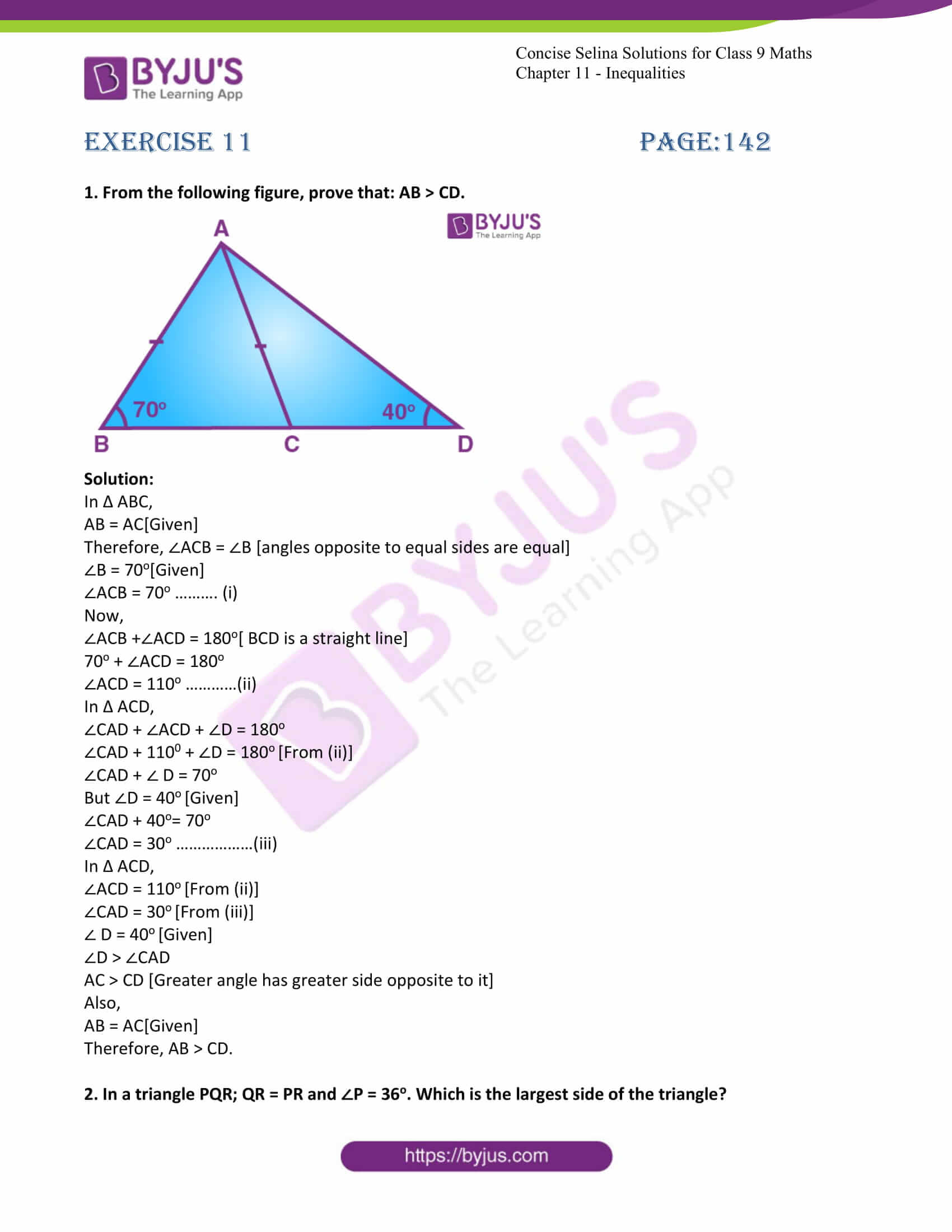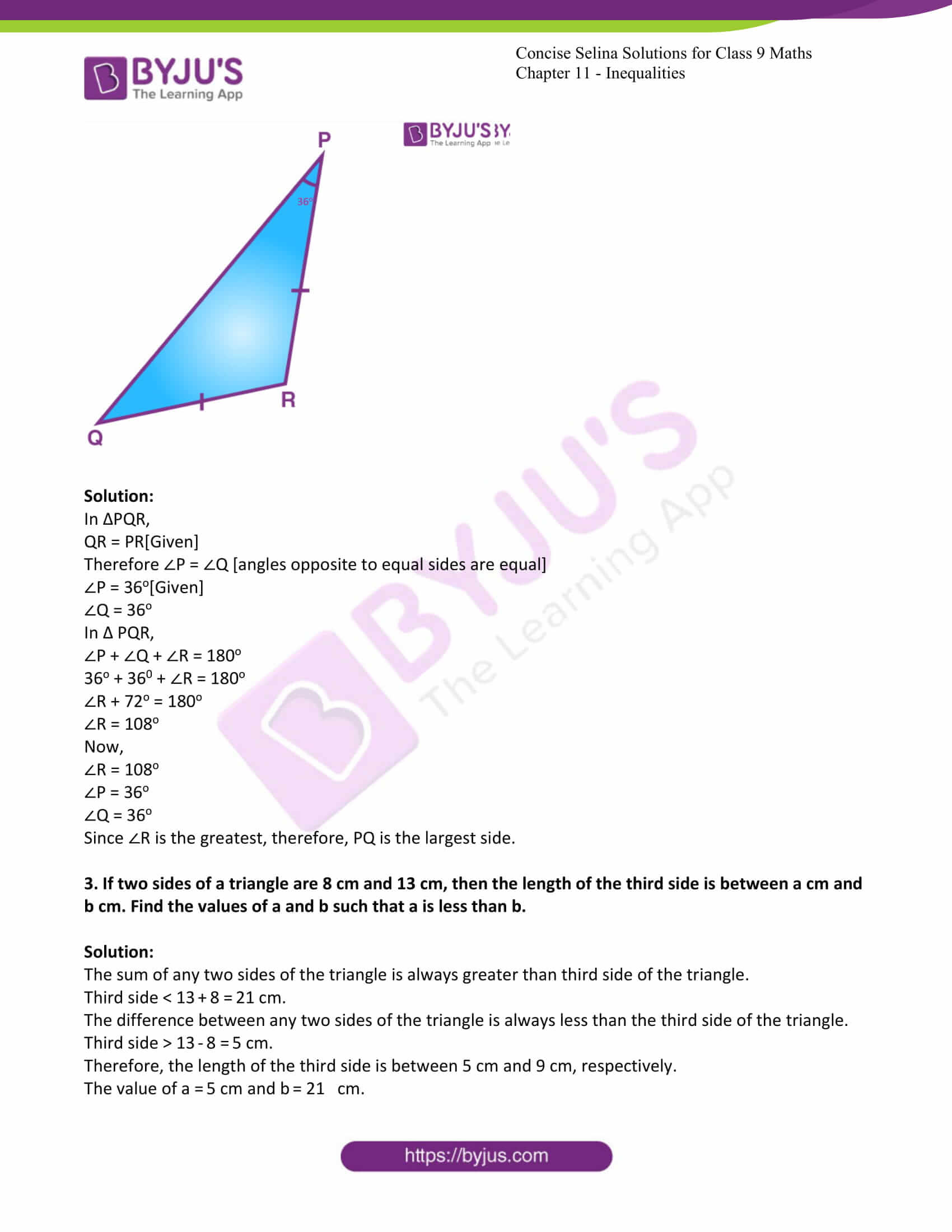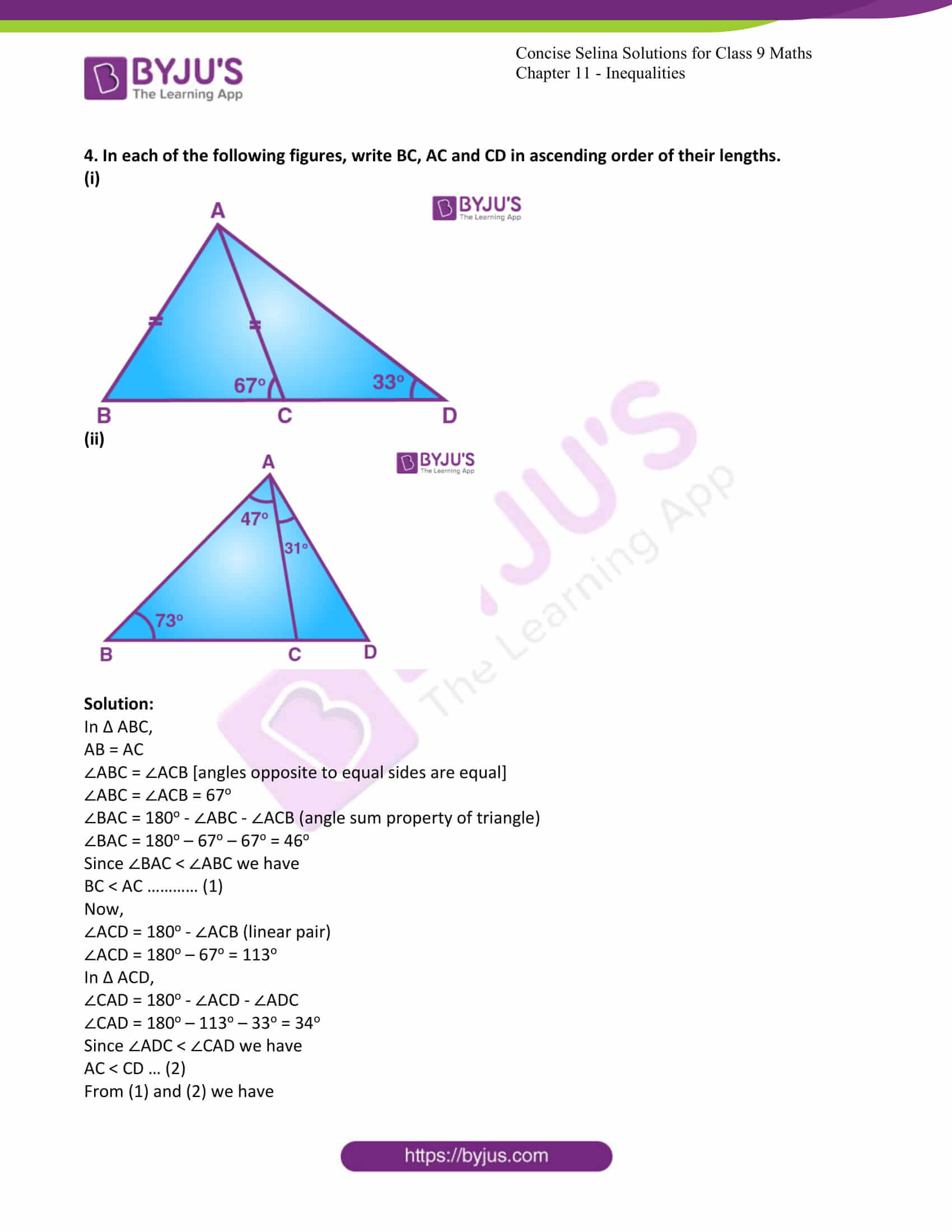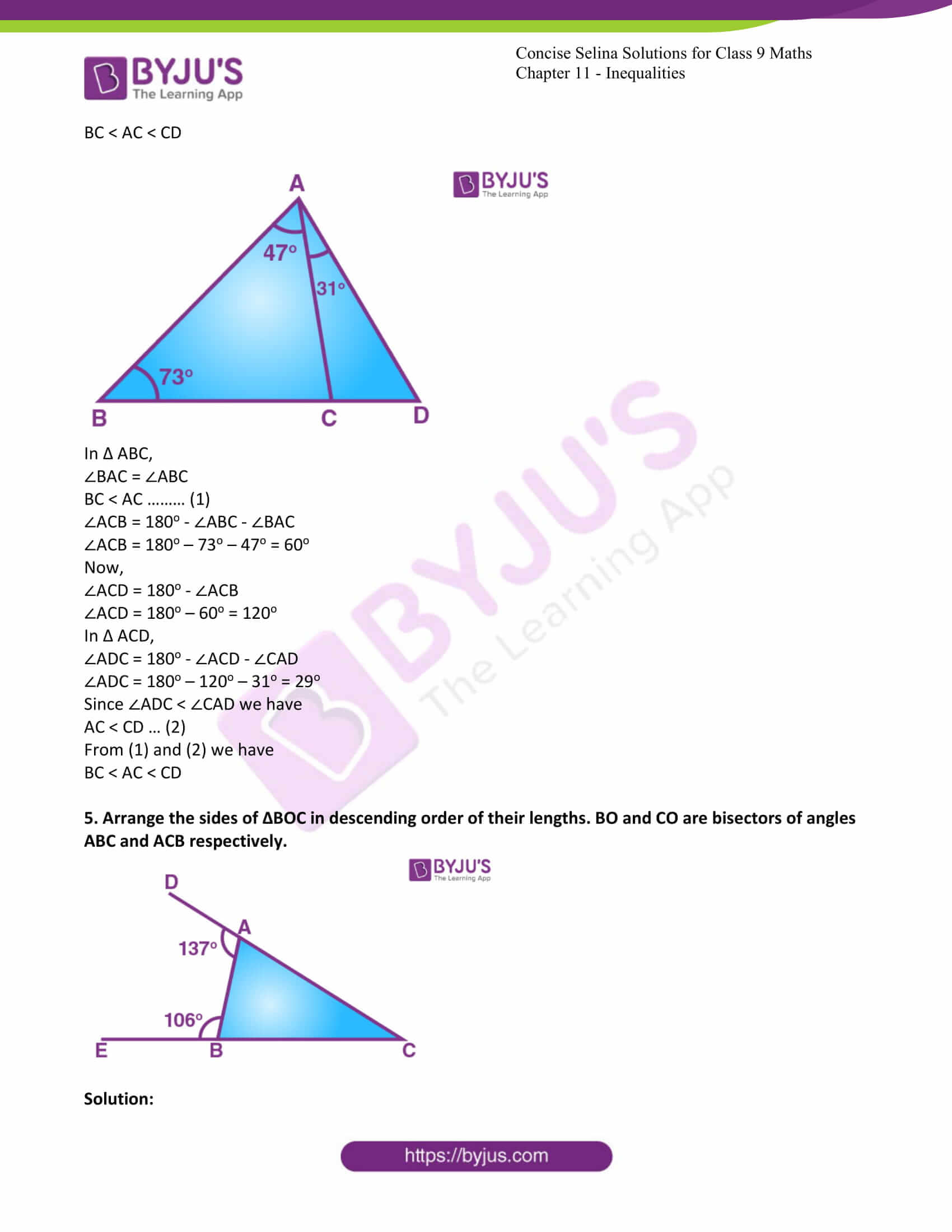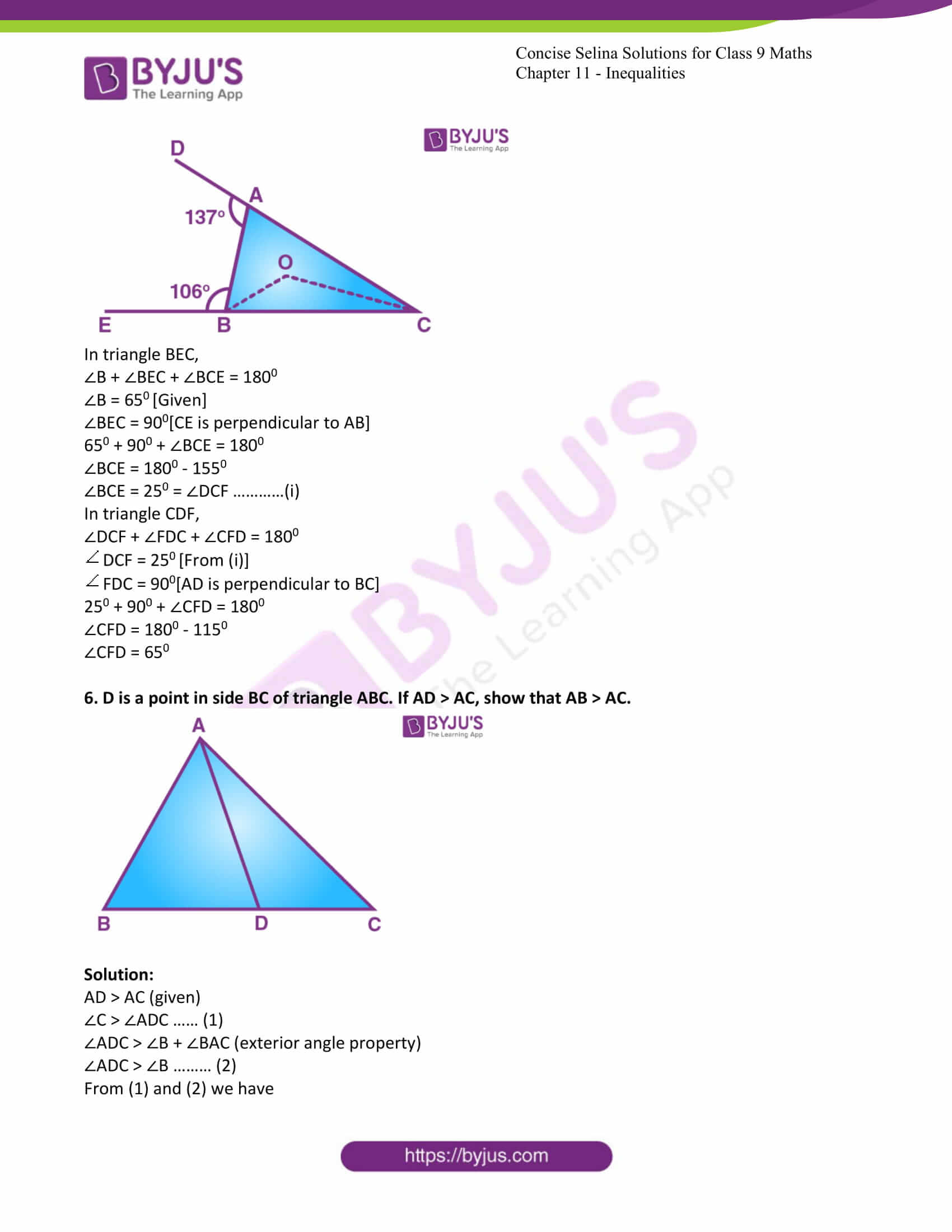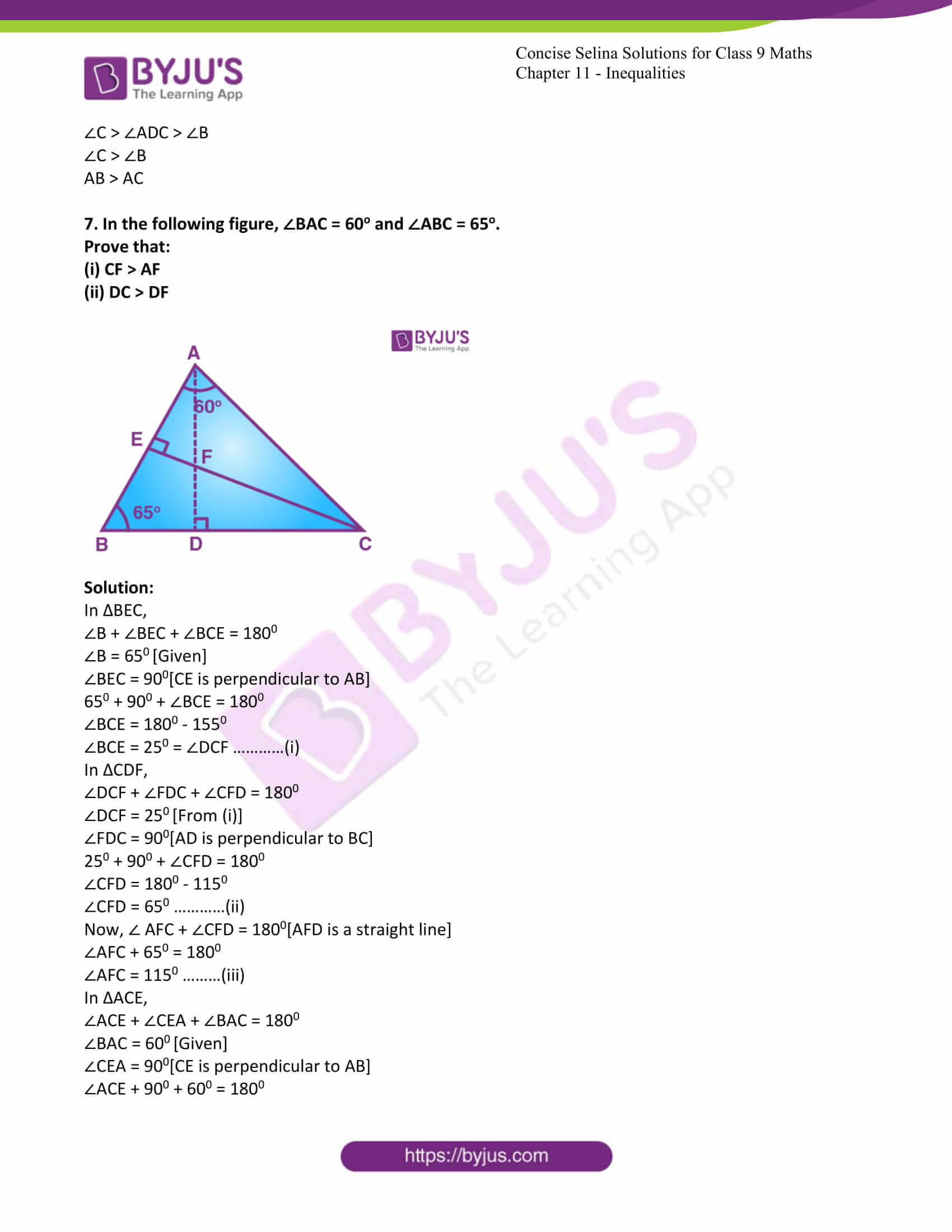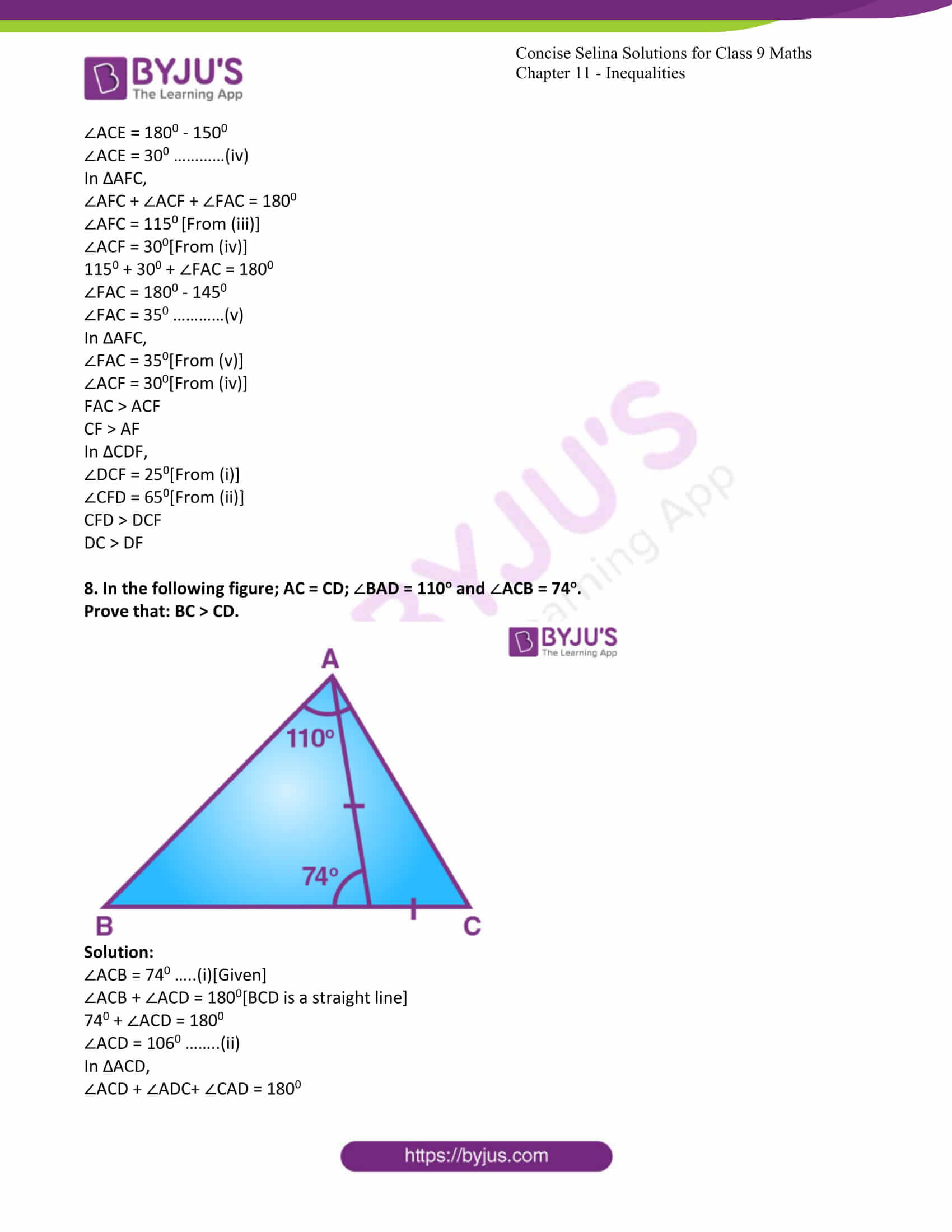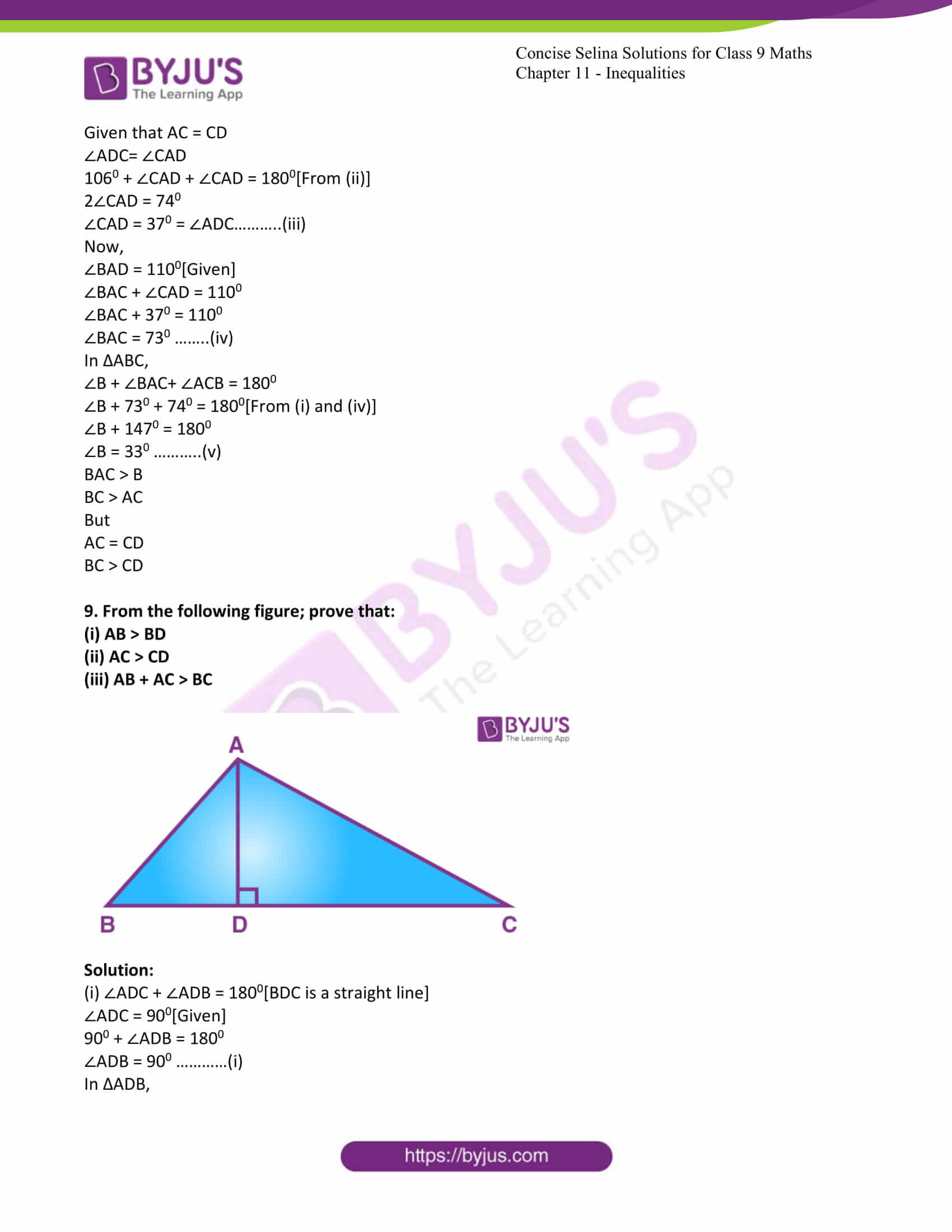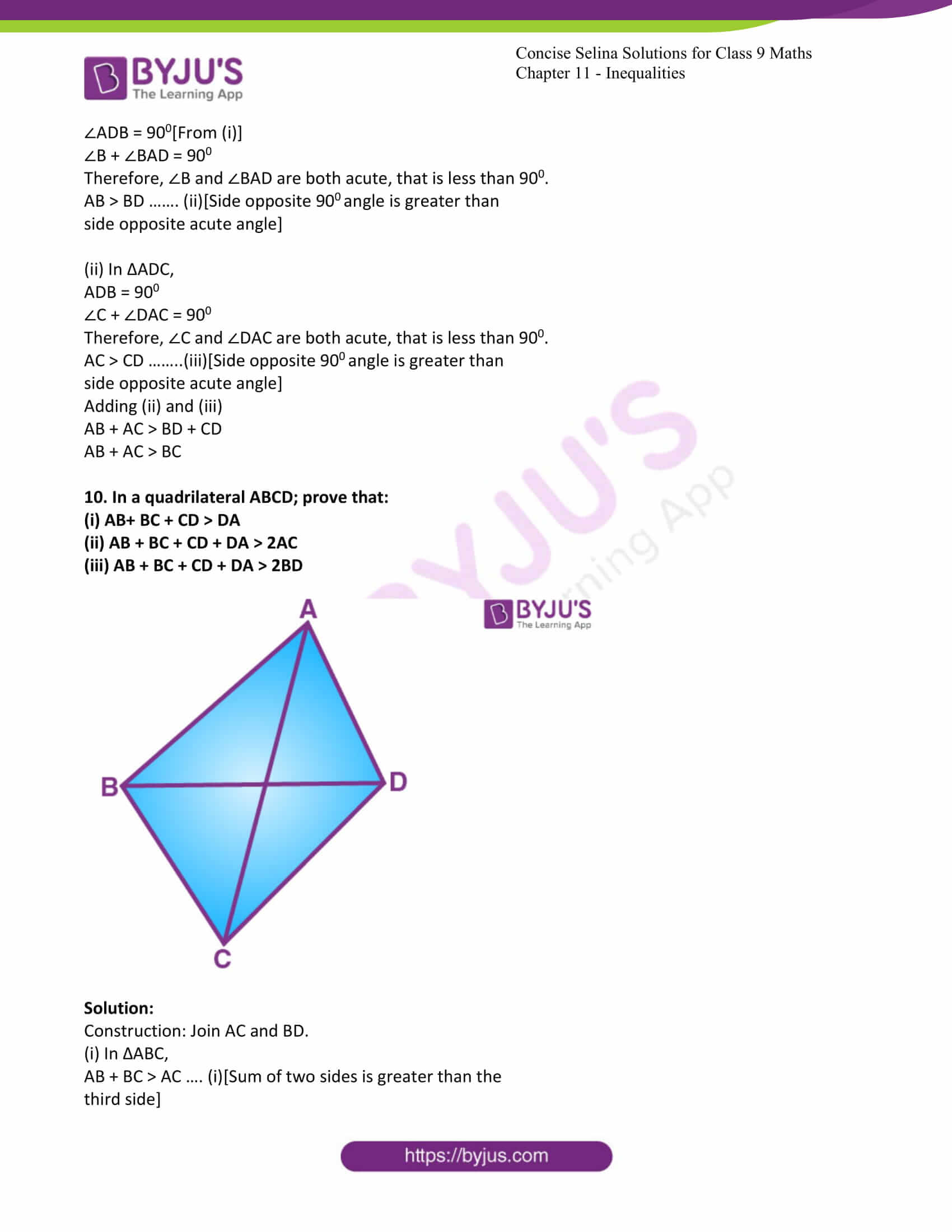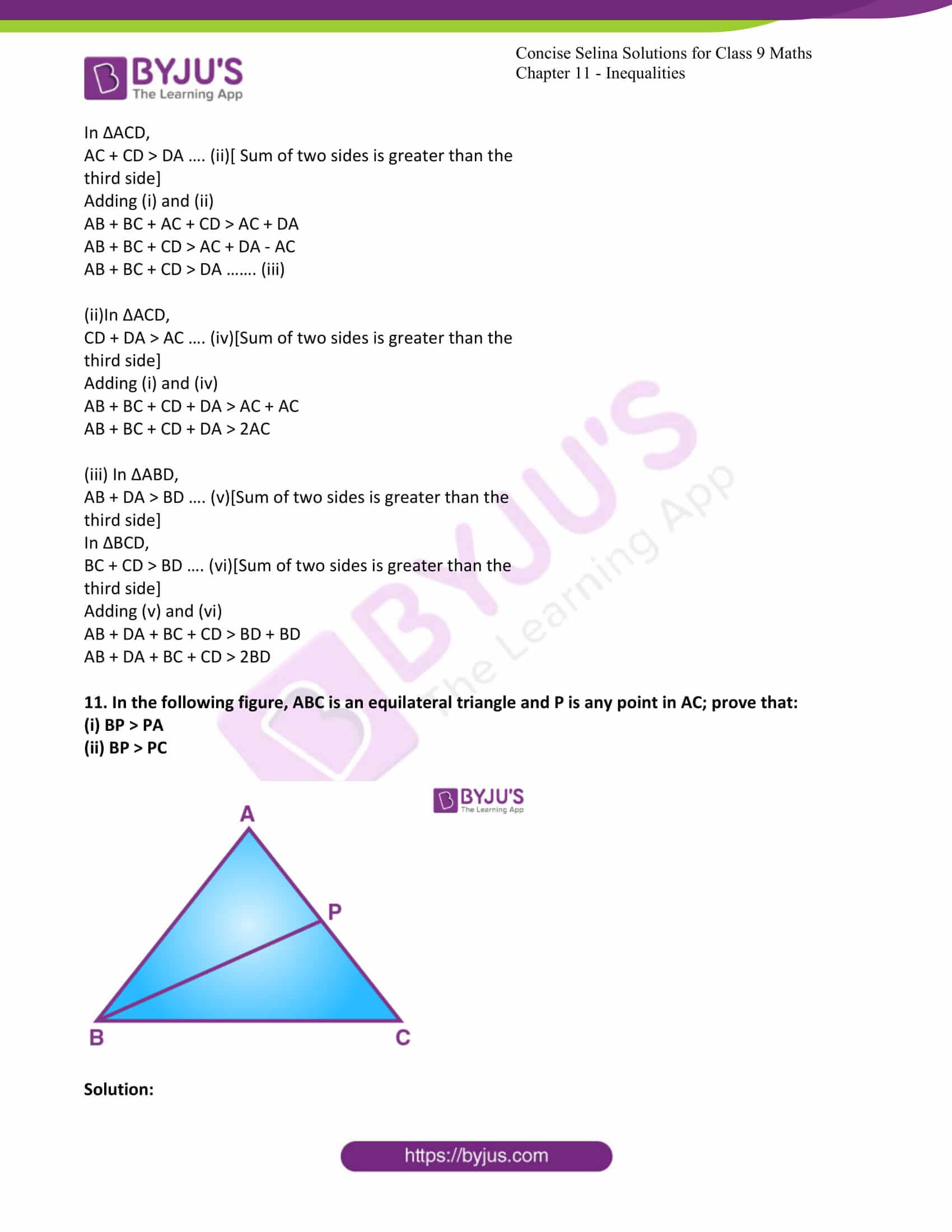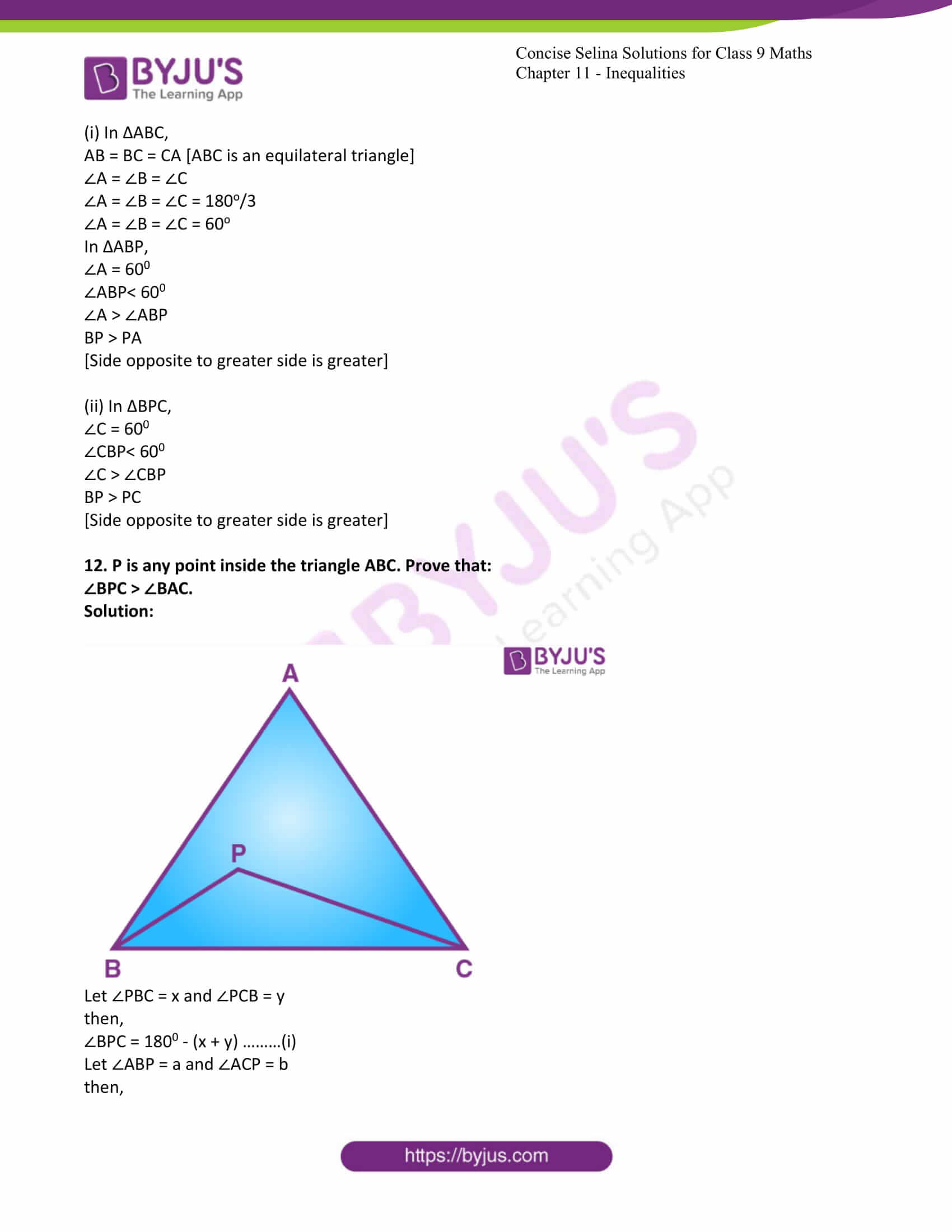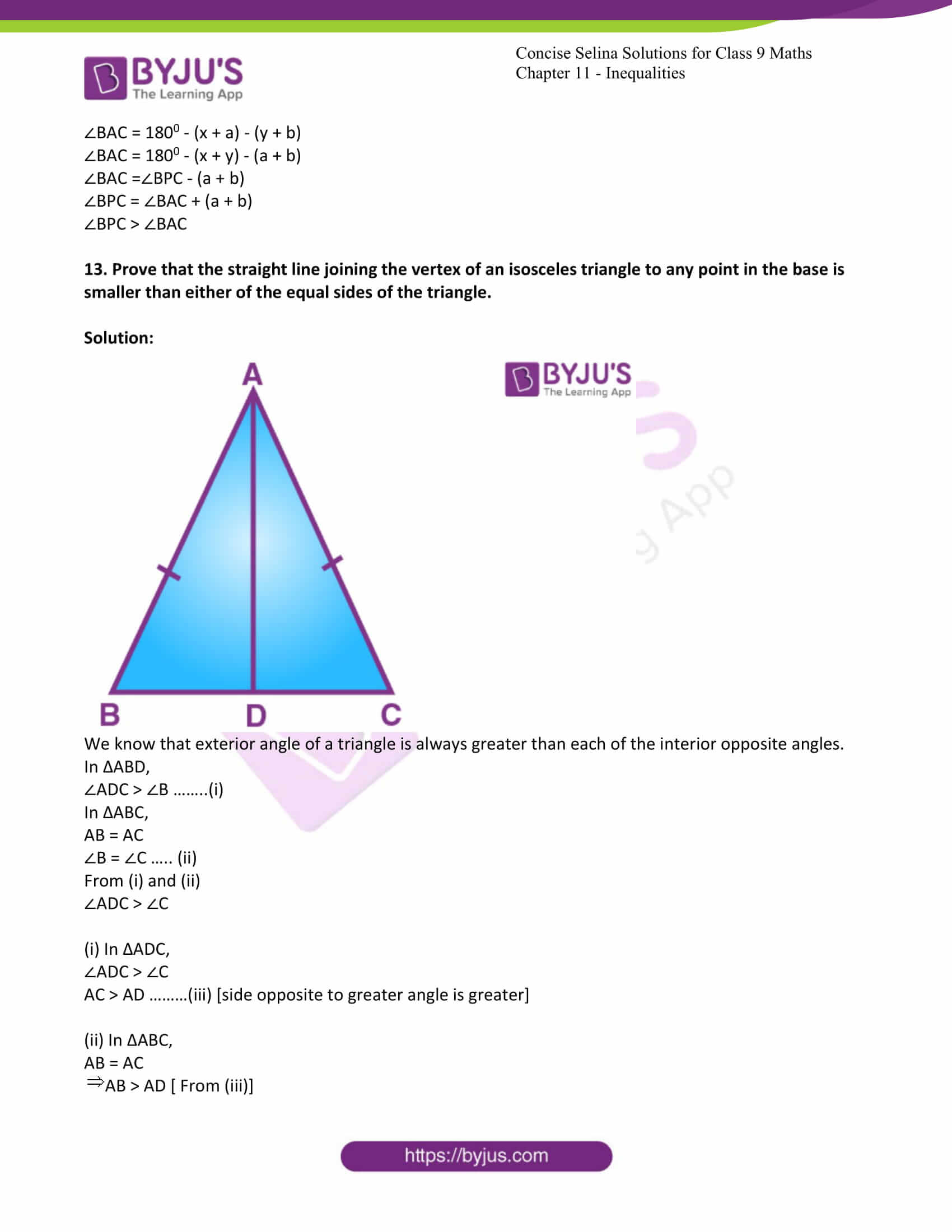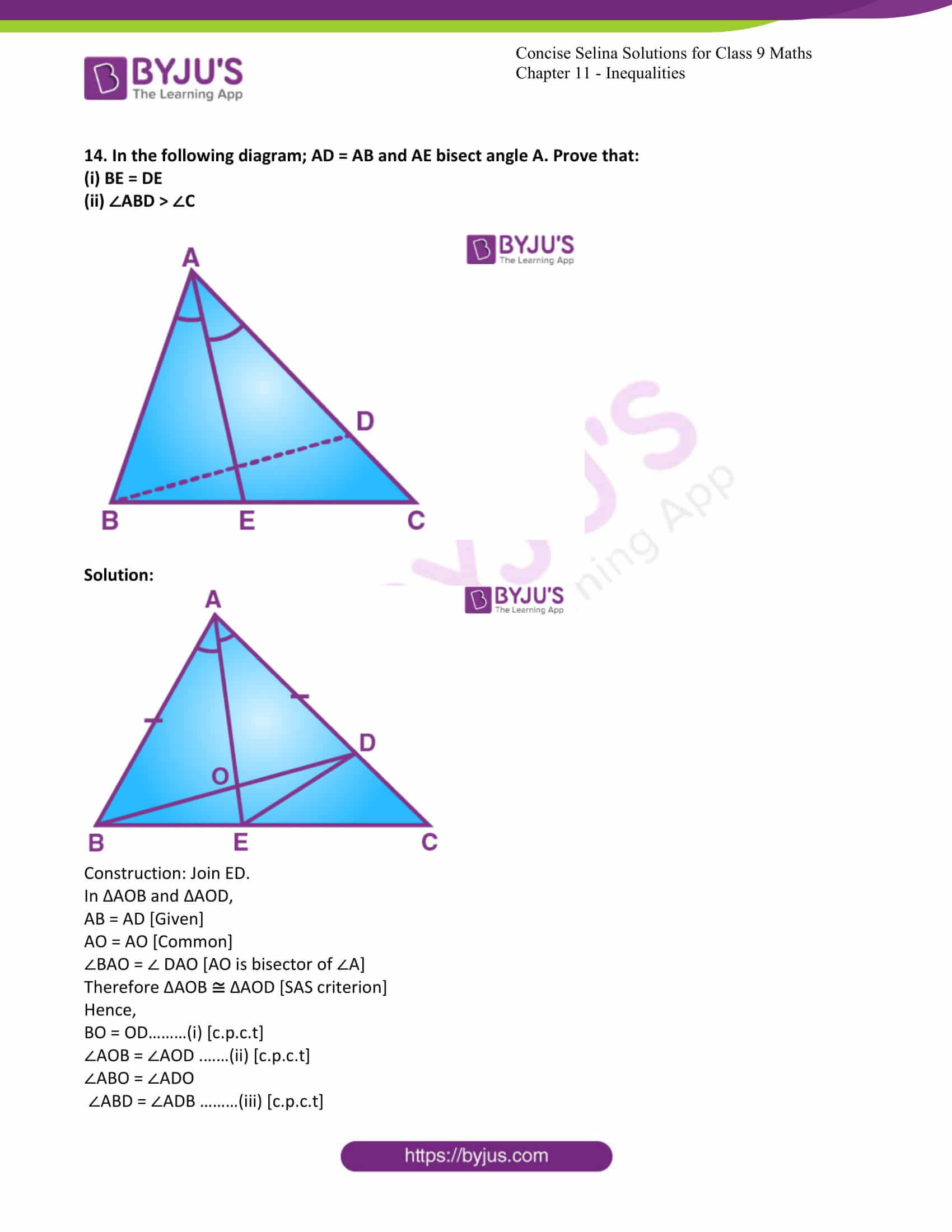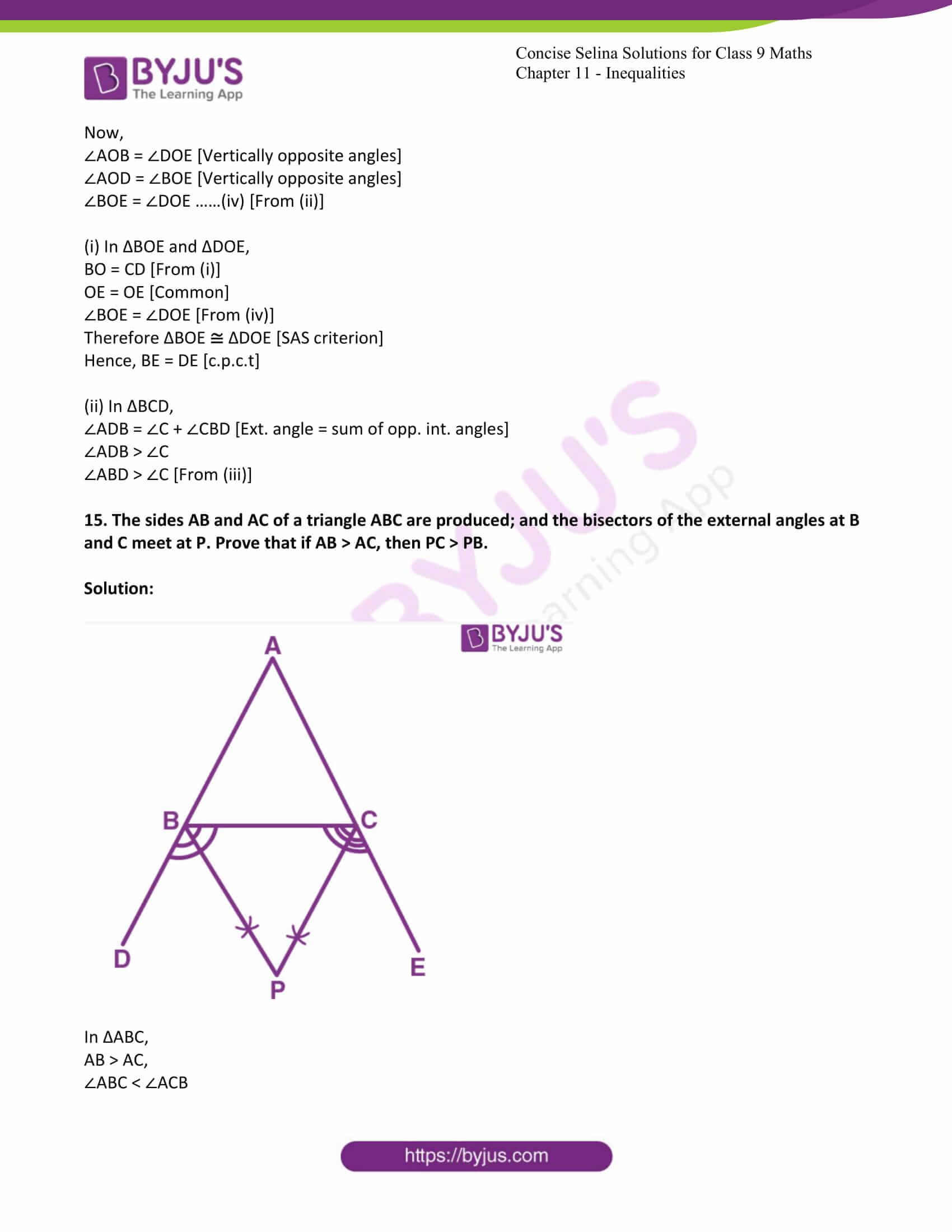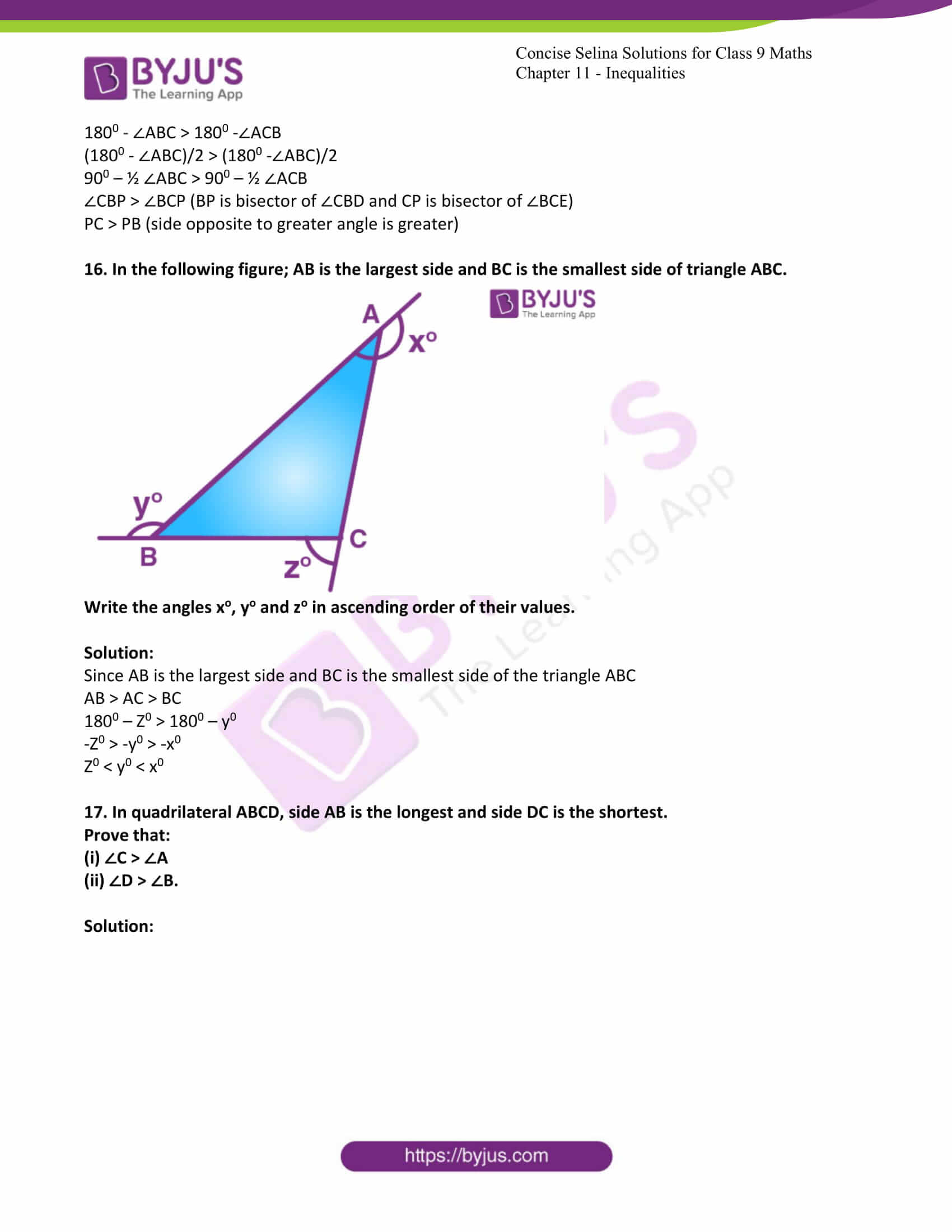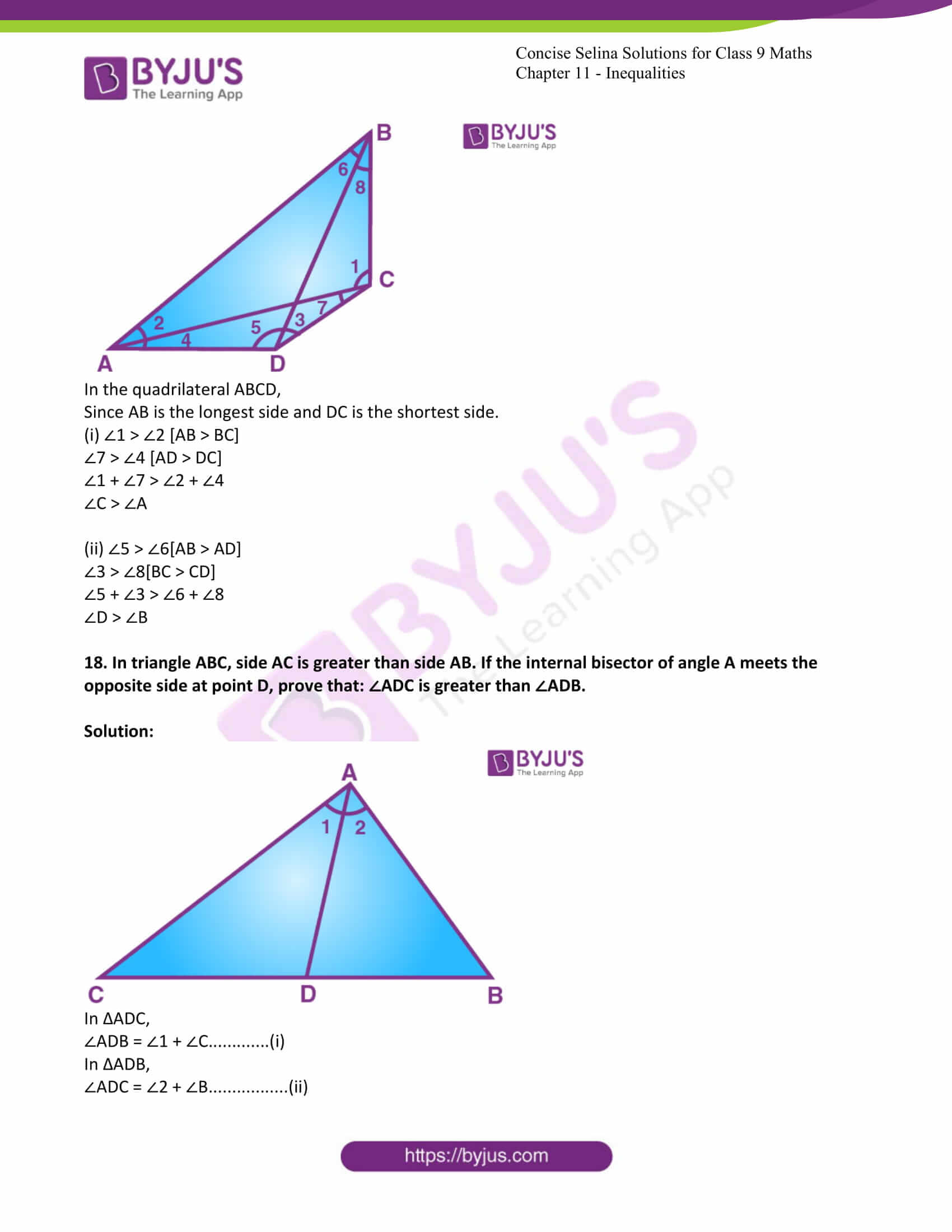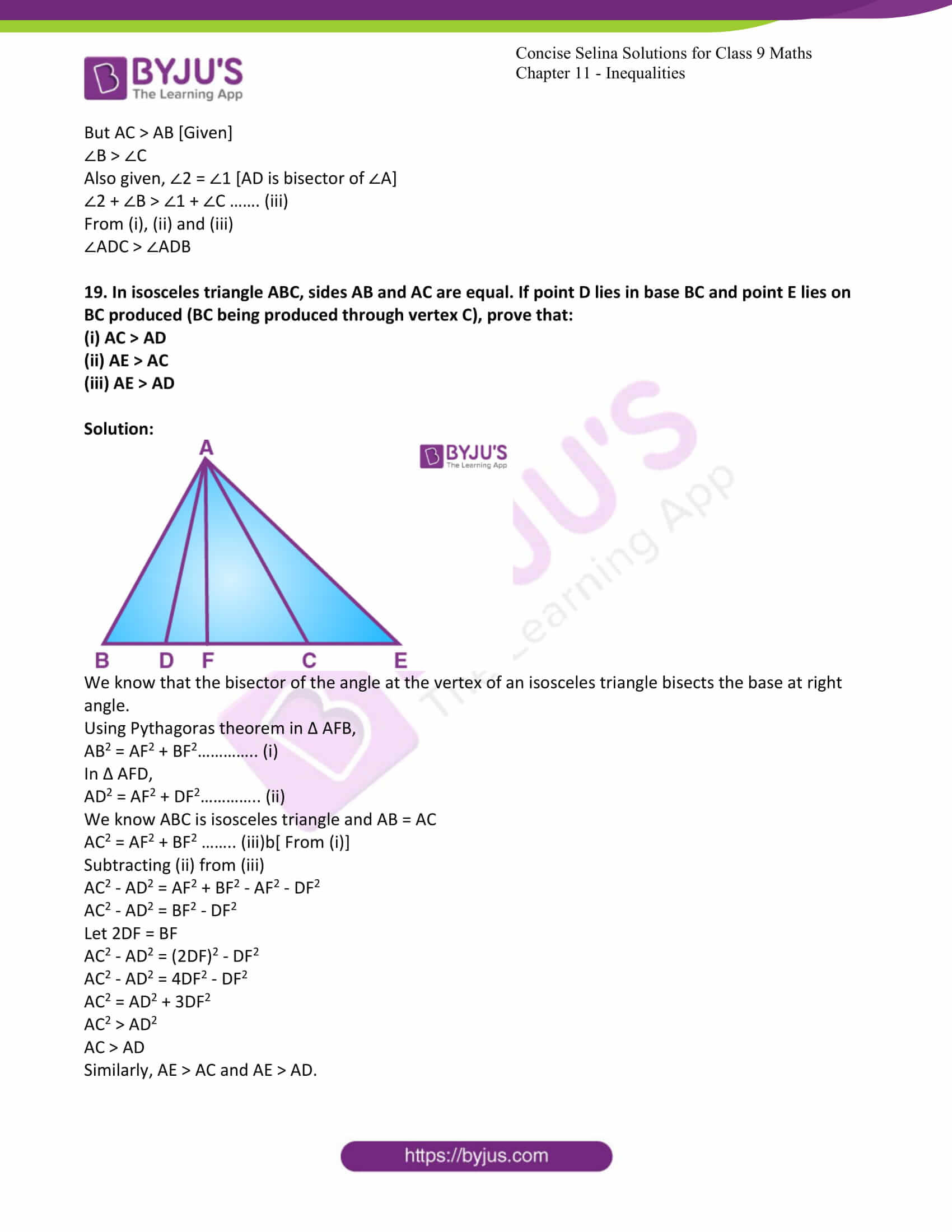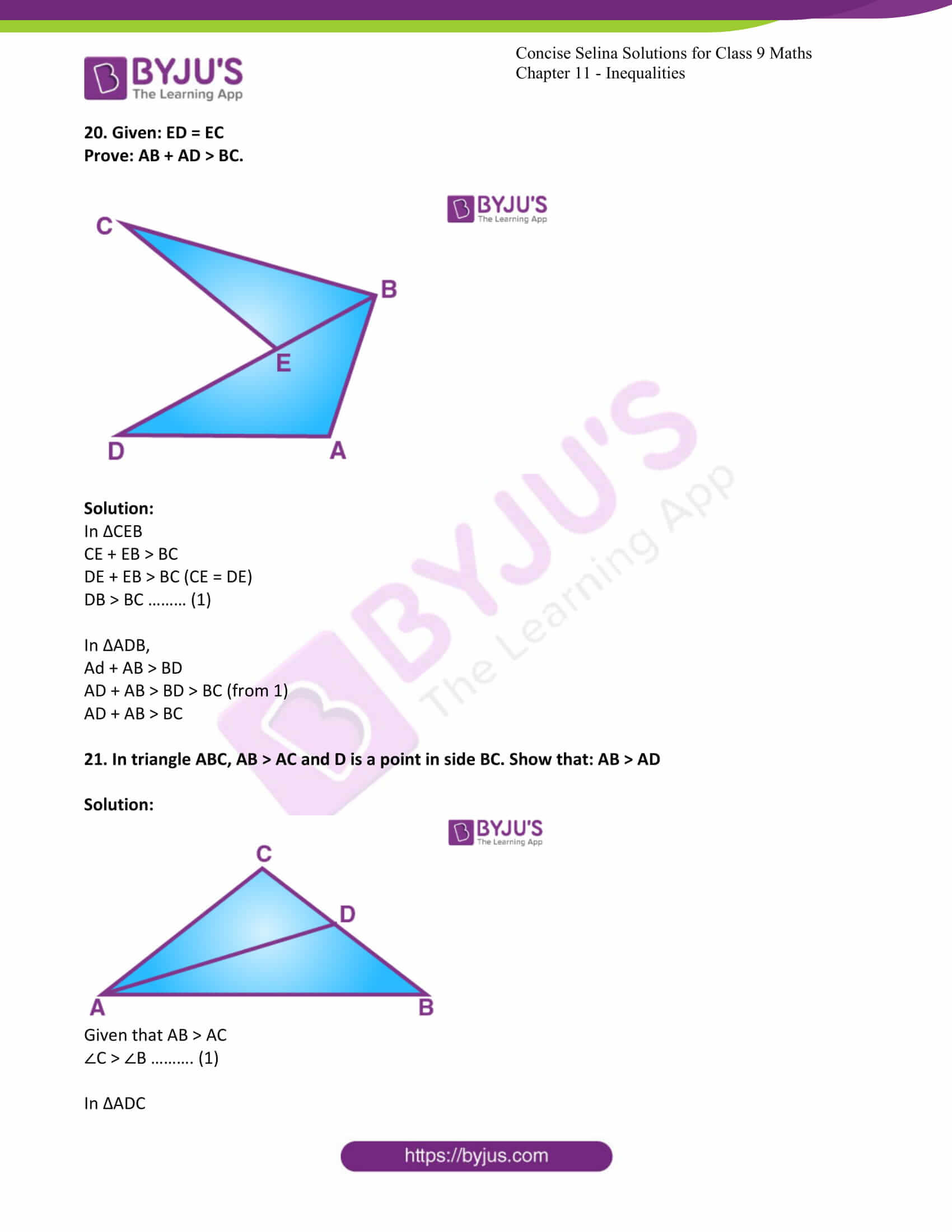Exercise 11 PAGE:142

1. From the following figure, prove that: AB > CD.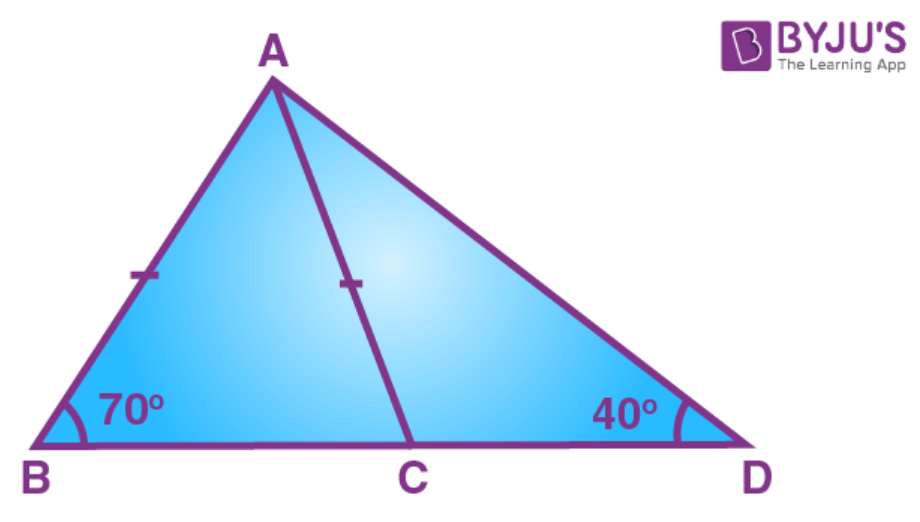Solution:

In ∆ ABC,

AB = AC[Given]

Therefore, ∠ACB = ∠B [angles opposite to equal sides are equal]

∠B = 70o[Given]

∠ACB = 70o ………. (i)

Now,

∠ACB +∠ACD = 180o[ BCD is a straight line]

70o + ∠ACD = 180o

∠ACD = 110o …………(ii)

In ∆ ACD,

∠CAD + ∠ACD + ∠D = 180o

∠CAD + 1100 + ∠D = 180[From (ii)]

∠CAD + ∠ D = 70o

But ∠D = 40[Given]

In ∆ ACD,

∠ACD = 110o [From (ii)]

∠ D = 40[Given]

AC > CD [Greater angle has greater side opposite to it]

Also,

AB = AC[Given]

Therefore, AB > CD.

2. In a triangle PQR; QR = PR and ∠P = 36o. Which is the largest side of the triangle?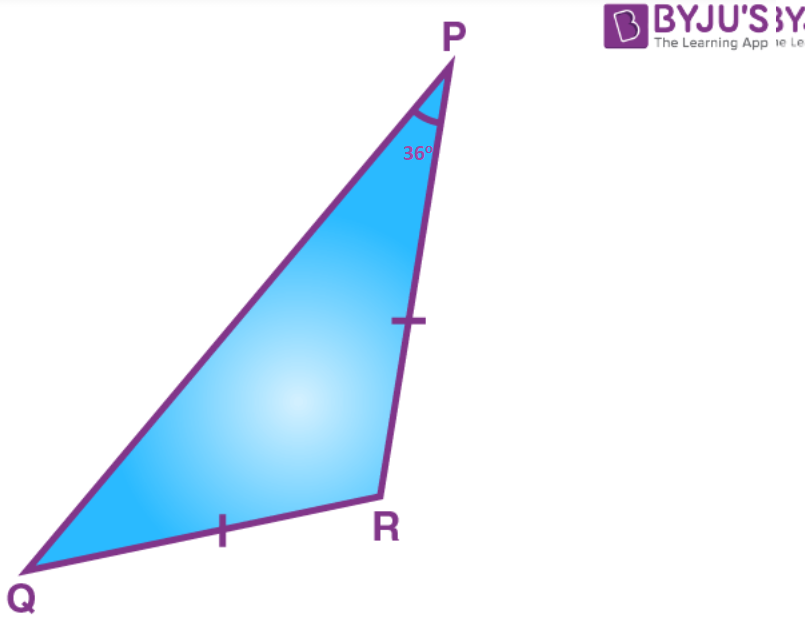Solution:

In ∆PQR,

QR = PR[Given]

Therefore ∠P = ∠Q [angles opposite to equal sides are equal]

∠P = 36o[Given]

∠Q = 36o

In ∆ PQR,

∠P + ∠Q + ∠R = 180o

36o + 360 + ∠R = 180o

∠R + 72o = 180o

∠R = 108o

Now,

∠R = 108o

∠P = 36o

∠Q = 36o

Since ∠R is the greatest, therefore, PQ is the largest side.

3. If two sides of a triangle are 8 cm and 13 cm, then the length of the third side is between a cm and b cm. Find the values of a and b such that a is less than b.

Solution:

The sum of any two sides of the triangle is always greater than third side of the triangle.

Third side < 13 + 8 = 21 cm.

The difference between any two sides of the triangle is always less than the third side of the triangle.

Third side > 13 8 = 5 cm.

Therefore, the length of the third side is between 5 cm and 9 cm, respectively.

The value of a = 5 cm and b = 21cm.

4. In each of the following figures, write BC, AC and CD in ascending order of their lengths.

(i)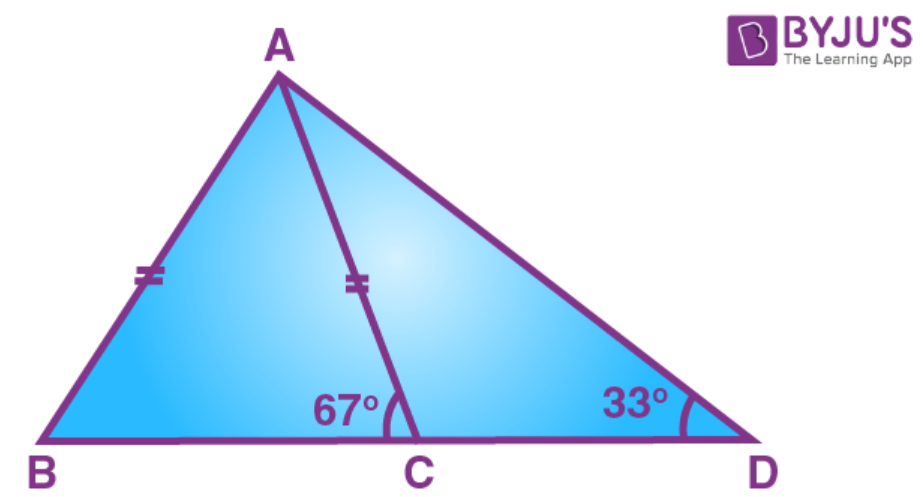(ii)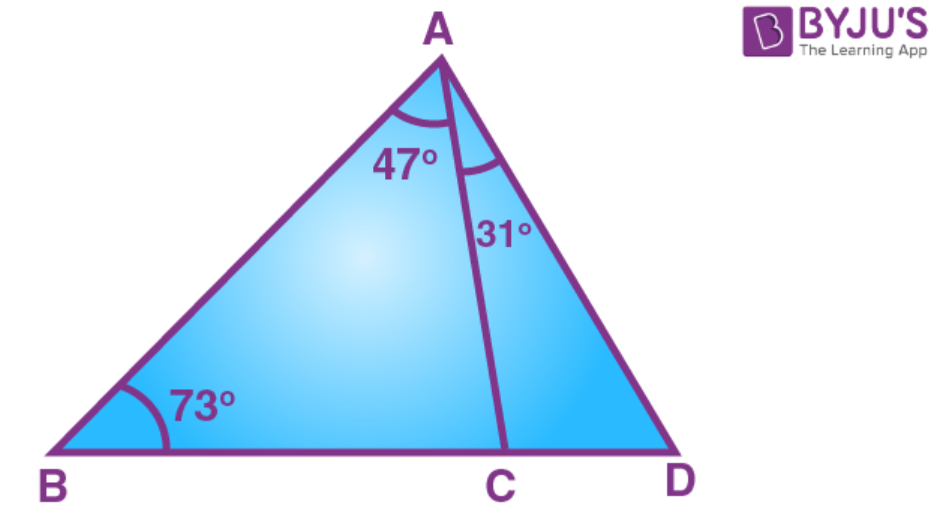Solution:

In ∆ ABC,

AB = AC

∠ABC = ∠ACB [angles opposite to equal sides are equal]

∠ABC = ∠ACB = 67o

∠BAC = 180o – ∠ABC – ∠ACB (angle sum property of triangle)

∠BAC = 180o – 67o – 67o = 46o

Since ∠BAC < ∠ABC we have

BC < AC ………… (1)

Now,

∠ACD = 180o – ∠ACB (linear pair)

∠ACD = 180o – 67o = 113o

In ∆ ACD,

∠CAD = 180o – 113o – 33o = 34o

AC < CD … (2)

From (1) and (2) we have

BC < AC < CD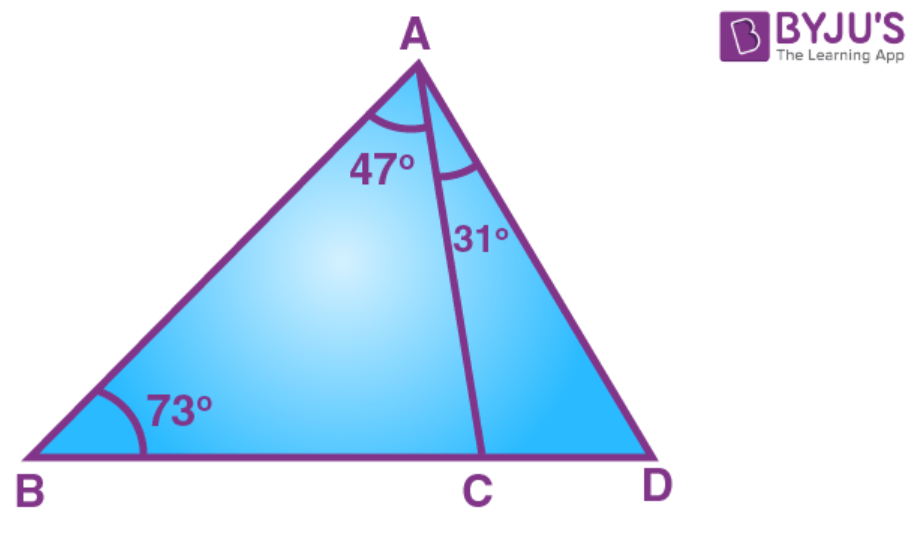In ∆ ABC,

∠BAC = ∠ABC

BC < AC ……… (1)

∠ACB = 180o – ∠ABC – ∠BAC

∠ACB = 180o – 73o – 47o = 60o

Now,

∠ACD = 180o – ∠ACB

∠ACD = 180o – 60o = 120o

In ∆ ACD,

∠ADC = 180o – 120o – 31o = 29o

AC < CD … (2)

From (1) and (2) we have

BC < AC < CD

5. Arrange the sides of ∆BOC in descending order of their lengths. BO and CO are bisectors of angles ABC and ACB respectively.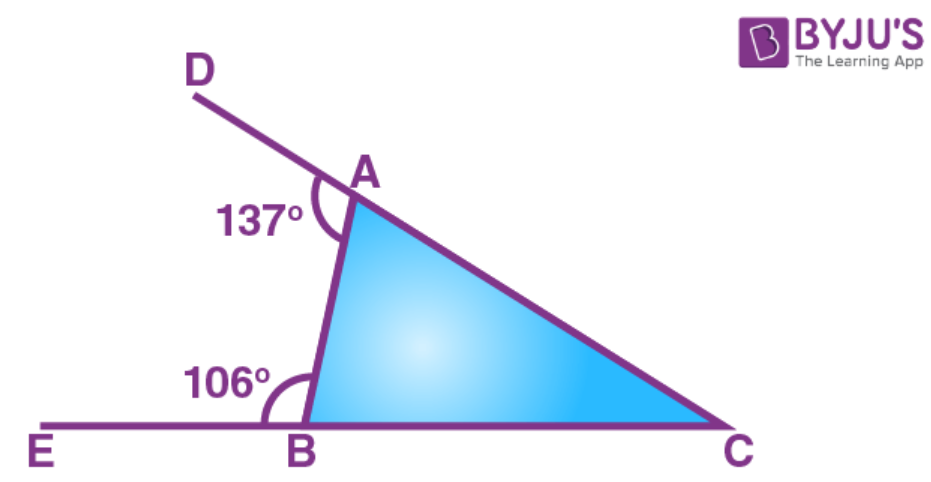Solution: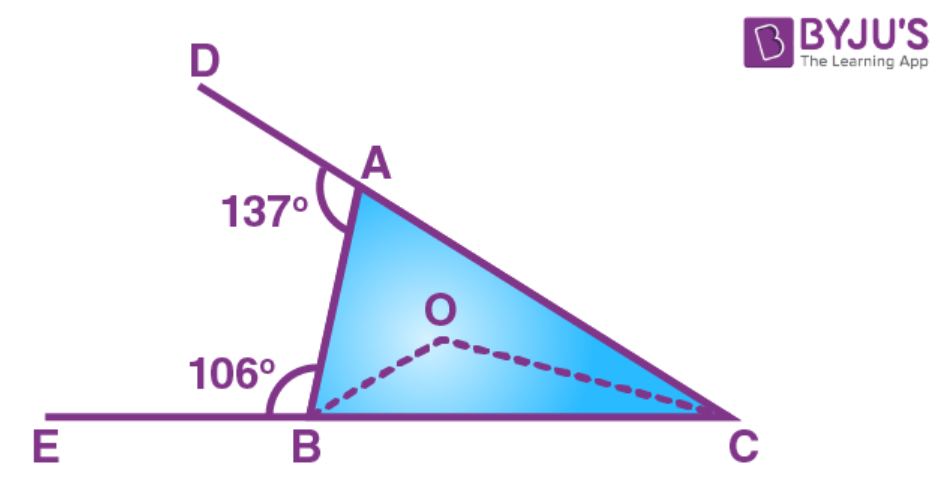In triangle BEC,

∠B + ∠BEC + ∠BCE = 1800

∠B = 65[Given]

∠BEC = 900[CE is perpendicular to AB]

650 + 900 + ∠BCE = 1800

∠BCE = 1800 – 1550

∠BCE = 250 = ∠DCF …………(i)

In triangle CDF,

∠DCF + ∠FDC + ∠CFD = 1800

∠DCF = 25[From (i)]

∠FDC = 900[AD is perpendicular to BC]

250 + 900 + ∠CFD = 1800

∠CFD = 1800 – 1150

∠CFD = 650

6. D is a point in side BC of triangle ABC. If AD > AC, show that AB > AC.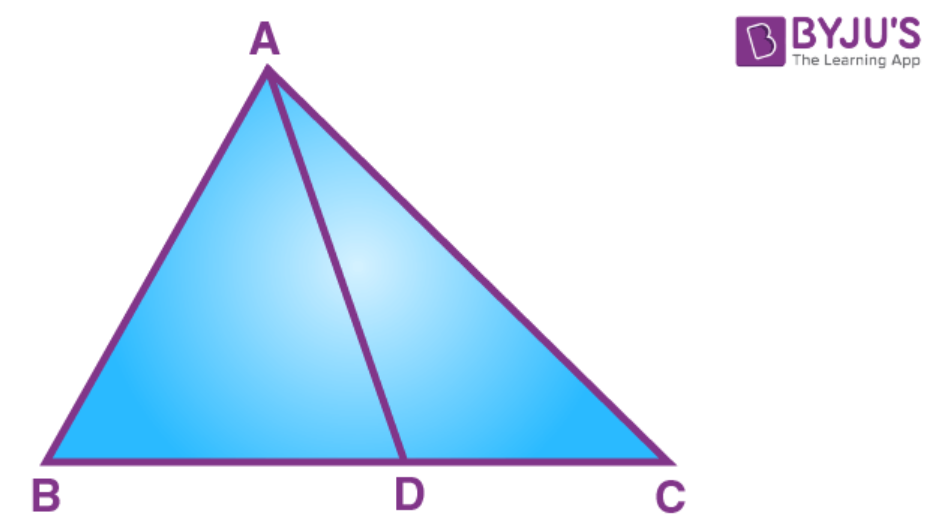Solution:

∠ADC > ∠B + ∠BAC (exterior angle property)

From (1) and (2) we have

∠C > ∠B

AB > AC

7. In the following figure, ∠BAC = 60o and ∠ABC = 65o.

Prove that:

(i) CF > AF

(ii) DC > DF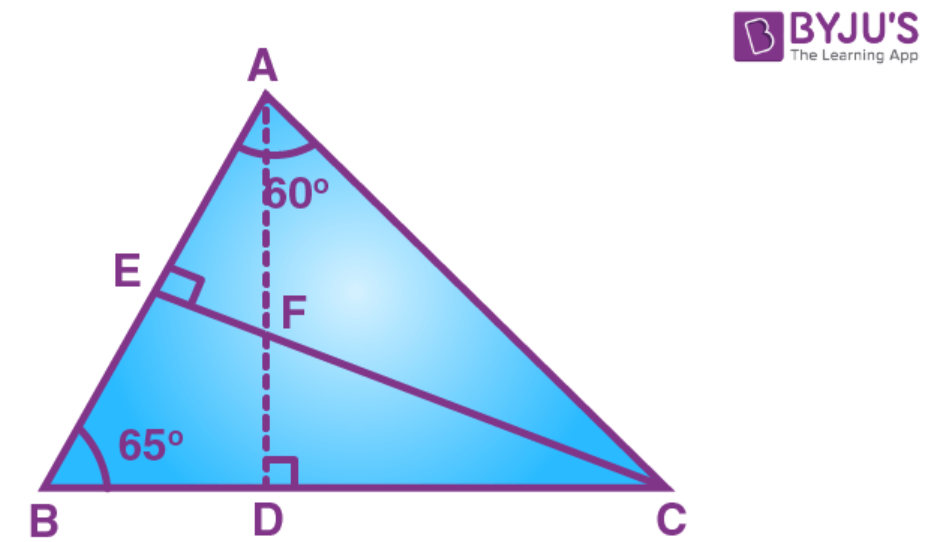Solution:

In ∆BEC,

∠B + ∠BEC + ∠BCE = 1800

∠B = 65[Given]

∠BEC = 900[CE is perpendicular to AB]

650 + 900 + ∠BCE = 1800

∠BCE = 1800 – 1550

∠BCE = 250 = ∠DCF …………(i)

In ∆CDF,

∠DCF + ∠FDC + ∠CFD = 1800

∠DCF = 25[From (i)]

∠FDC = 900[AD is perpendicular to BC]

250 + 900 + ∠CFD = 1800

∠CFD = 1800 – 1150

∠CFD = 650 …………(ii)

Now, ∠ AFC + ∠CFD = 1800[AFD is a straight line]

∠AFC + 650 = 1800

∠AFC = 1150 ………(iii)

In ∆ACE,

∠ACE + ∠CEA + ∠BAC = 1800

∠BAC = 60[Given]

∠CEA = 900[CE is perpendicular to AB]

∠ACE + 900 + 600 = 1800

∠ACE = 1800 – 1500

∠ACE = 300 …………(iv)

In ∆AFC,

∠AFC + ∠ACF + ∠FAC = 1800

∠AFC = 115[From (iii)]

∠ACF = 300[From (iv)]

1150 + 300 + ∠FAC = 1800

∠FAC = 1800 – 1450

∠FAC = 350 …………(v)

In ∆AFC,

∠FAC = 350[From (v)]

∠ACF = 300[From (iv)]

FAC > ACF

CF > AF

In ∆CDF,

∠DCF = 250[From (i)]

∠CFD = 650[From (ii)]

CFD > DCF

DC > DF

8. In the following figure; AC = CD; BAD = 110o and ACB = 74o.

Prove that: BC > CD.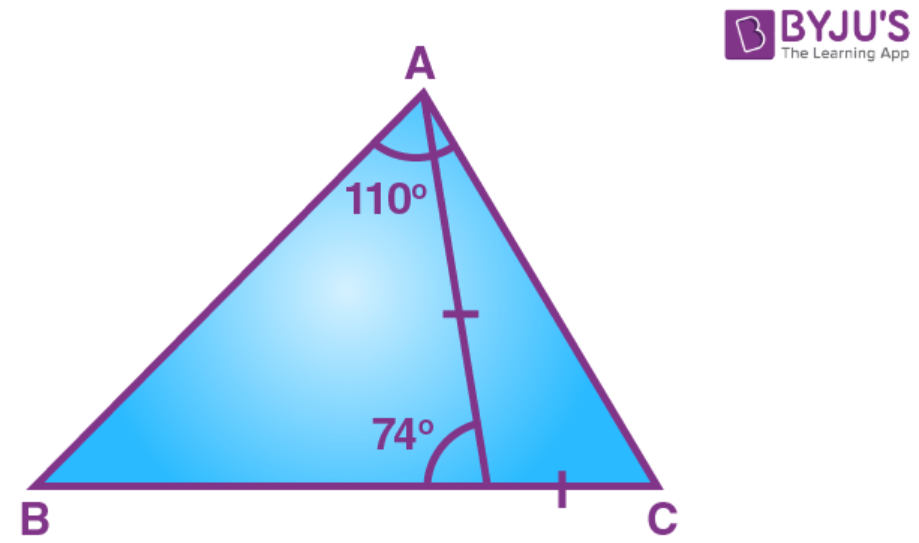Solution:

∠ACB = 740 …..(i)[Given]

∠ACB + ∠ACD = 1800[BCD is a straight line]

740 + ∠ACD = 1800

∠ACD = 1060 ……..(ii)

In ∆ACD,

Given that AC = CD

Now,

∠BAC + 370 = 1100

∠BAC = 730 ……..(iv)

In ∆ABC,

∠B + ∠BAC+ ∠ACB = 1800

∠B + 730 + 740 = 1800[From (i) and (iv)]

∠B + 1470 = 1800

∠B = 330 ………..(v)

BAC > B

BC > AC

But

AC = CD

BC > CD

9. From the following figure; prove that:

(i) AB > BD

(ii) AC > CD

(iii) AB + AC > BC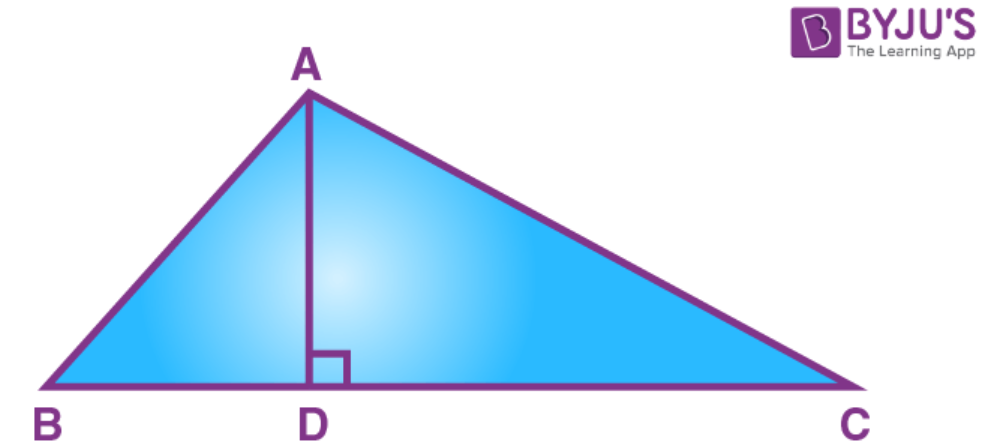Solution:

Therefore, ∠B and ∠BAD are both acute, that is less than 900.

AB > BD ……. (ii)[Side opposite 90angle is greater than

side opposite acute angle]

∠C + ∠DAC = 900

Therefore, ∠C and ∠DAC are both acute, that is less than 900.

AC > CD ……..(iii)[Side opposite 90angle is greater than

side opposite acute angle]

AB + AC > BD + CD

AB + AC > BC

10. In a quadrilateral ABCD; prove that:

(i) AB+ BC + CD > DA

(ii) AB + BC + CD + DA > 2AC

(iii) AB + BC + CD + DA > 2BD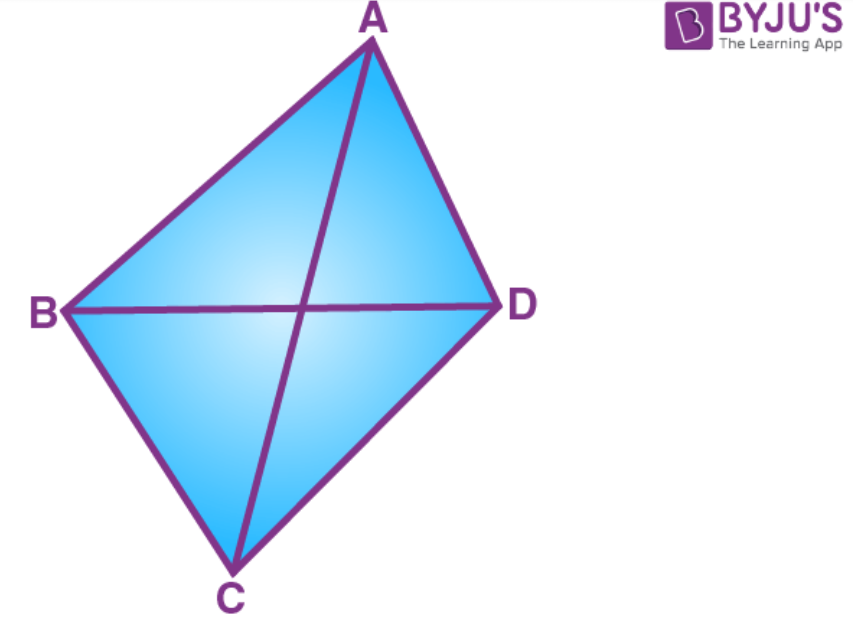Solution:

Construction: Join AC and BD.

(i) In ∆ABC,

AB + BC > AC …. (i)[Sum of two sides is greater than the

third side]

In ∆ACD,

AC + CD > DA …. (ii)[ Sum of two sides is greater than the

third side]

AB + BC + AC + CD > AC + DA

AB + BC + CD > AC + DA – AC

AB + BC + CD > DA ……. (iii)

(ii)In ∆ACD,

CD + DA > AC …. (iv)[Sum of two sides is greater than the

third side]

AB + BC + CD + DA > AC + AC

AB + BC + CD + DA > 2AC

(iii) In ∆ABD,

AB + DA > BD …. (v)[Sum of two sides is greater than the

third side]

In ∆BCD,

BC + CD > BD …. (vi)[Sum of two sides is greater than the

third side]

AB + DA + BC + CD > BD + BD

AB + DA + BC + CD > 2BD

11. In the following figure, ABC is an equilateral triangle and P is any point in AC; prove that:

(i) BP > PA

(ii) BP > PC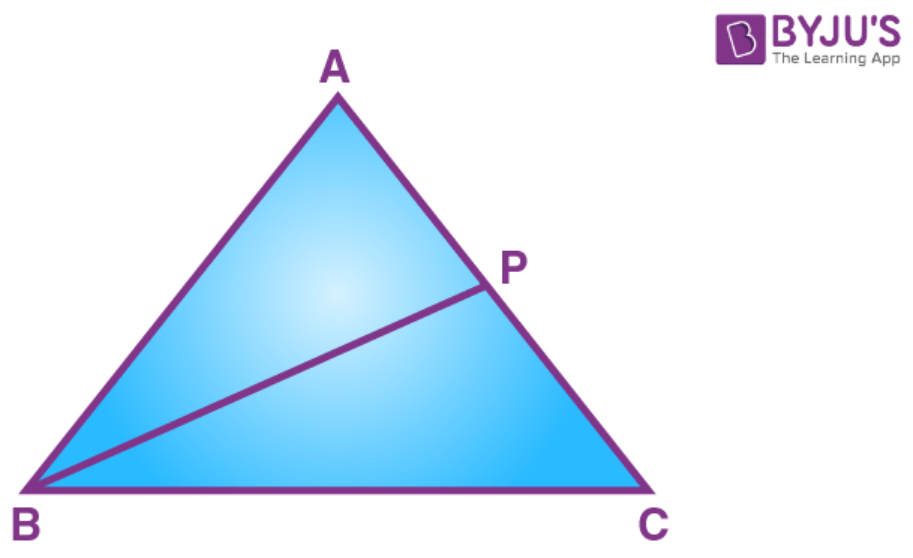Solution:

(i) In ∆ABC,

AB = BC = CA [ABC is an equilateral triangle]

∠A = ∠B = ∠C

∠A = ∠B = ∠C = 180o/3

∠A = ∠B = ∠C = 60o

In ∆ABP,

∠A = 600

∠ABP< 600

∠A > ∠ABP

BP > PA

[Side opposite to greater side is greater]

(ii) In ∆BPC,

∠C = 600

∠CBP< 600

∠C > ∠CBP

BP > PC

[Side opposite to greater side is greater]

12. P is any point inside the triangle ABC. Prove that:

∠BPC > ∠BAC.

Solution: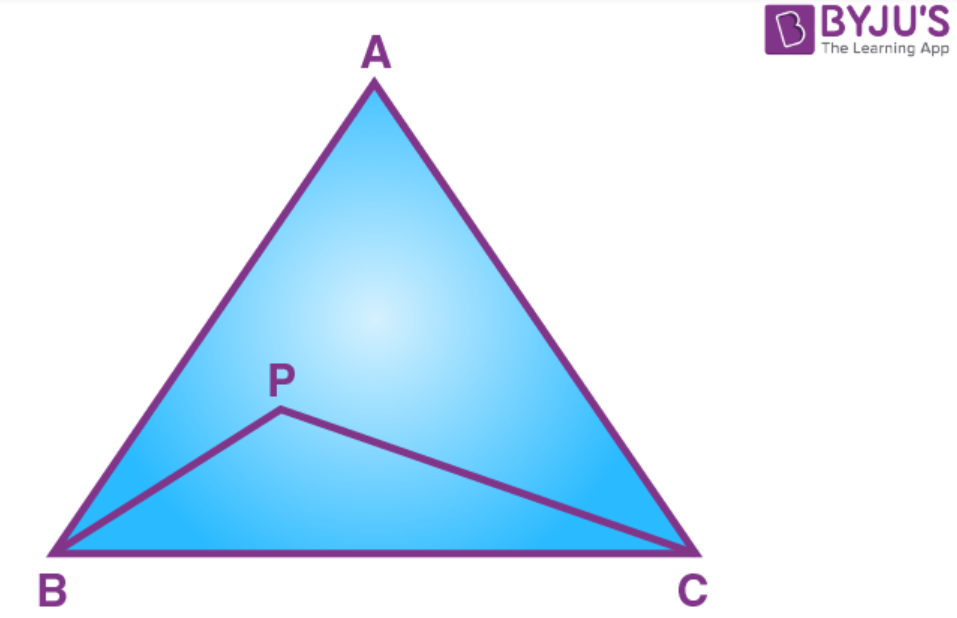Let ∠PBC = x and ∠PCB = y

then,

∠BPC = 1800 – (x + y) ………(i)

Let ∠ABP = a and ∠ACP = b

then,

∠BAC = 1800 – (x + a) – (y + b)

∠BAC = 1800 – (x + y) – (a + b)

∠BAC =∠BPC – (a + b)

∠BPC = ∠BAC + (a + b)

∠BPC > ∠BAC

13. Prove that the straight line joining the vertex of an isosceles triangle to any point in the base is smaller than either of the equal sides of the triangle.

Solution: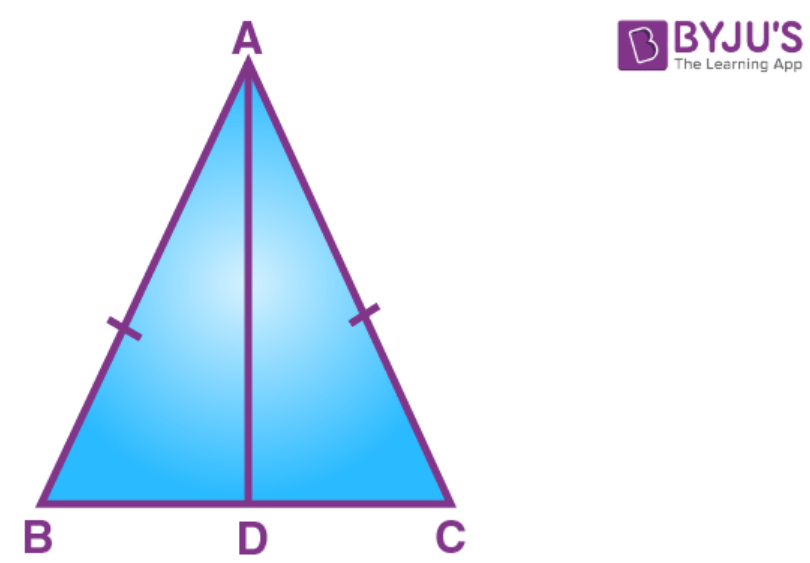We know that exterior angle of a triangle is always greater than each of the interior opposite angles.

In ∆ABD,

In ∆ABC,

AB = AC

∠B = ∠C ….. (ii)

From (i) and (ii)

AC > AD ………(iii) [side opposite to greater angle is greater]

(ii) In ∆ABC,

AB = AC

AB > AD [ From (iii)]

14. In the following diagram; AD = AB and AE bisect angle A. Prove that:

(i) BE = DE

(ii) ∠ABD > ∠C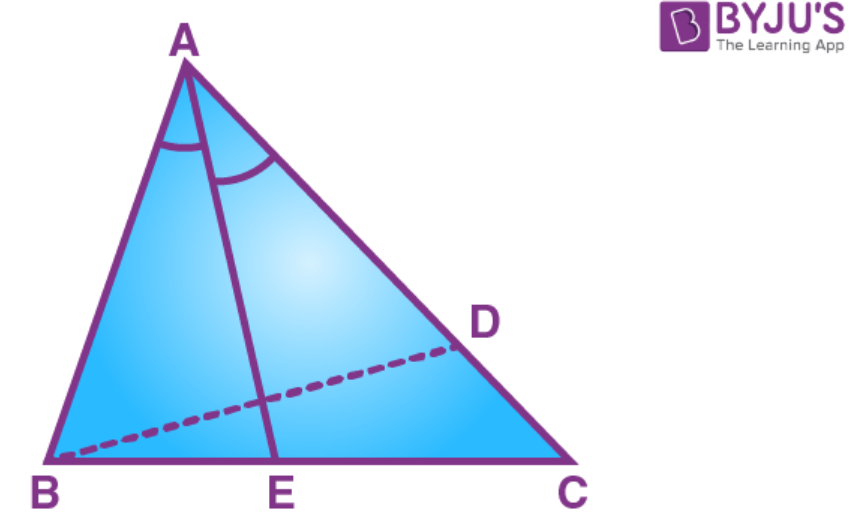Solution: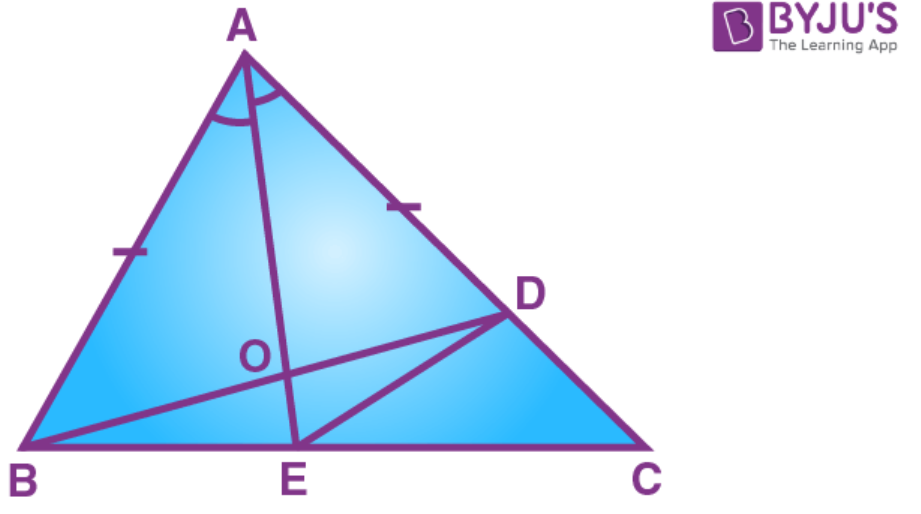Construction: Join ED.

In ∆AOB and ∆AOD,

AO = AO [Common]

∠BAO = ∠ DAO [AO is bisector of ∠A]

Therefore ∆AOB ∆AOD [SAS criterion]

Hence,

BO = OD………(i) [c.p.c.t]

∠AOB = ∠AOD .……(ii) [c.p.c.t]

Now,

∠AOB = ∠DOE [Vertically opposite angles]

∠AOD = ∠BOE [Vertically opposite angles]

∠BOE = ∠DOE ……(iv) [From (ii)]

(i) In ∆BOE and ∆DOE,

BO = CD [From (i)]

OE = OE [Common]

∠BOE = ∠DOE [From (iv)]

Therefore ∆BOE ∆DOE [SAS criterion]

Hence, BE = DE [c.p.c.t]

(ii) In ∆BCD,

∠ADB = ∠C + ∠CBD [Ext. angle = sum of opp. int. angles]

∠ABD > ∠C [From (iii)]

15. The sides AB and AC of a triangle ABC are produced; and the bisectors of the external angles at B and C meet at P. Prove that if AB > AC, then PC > PB.

Solution: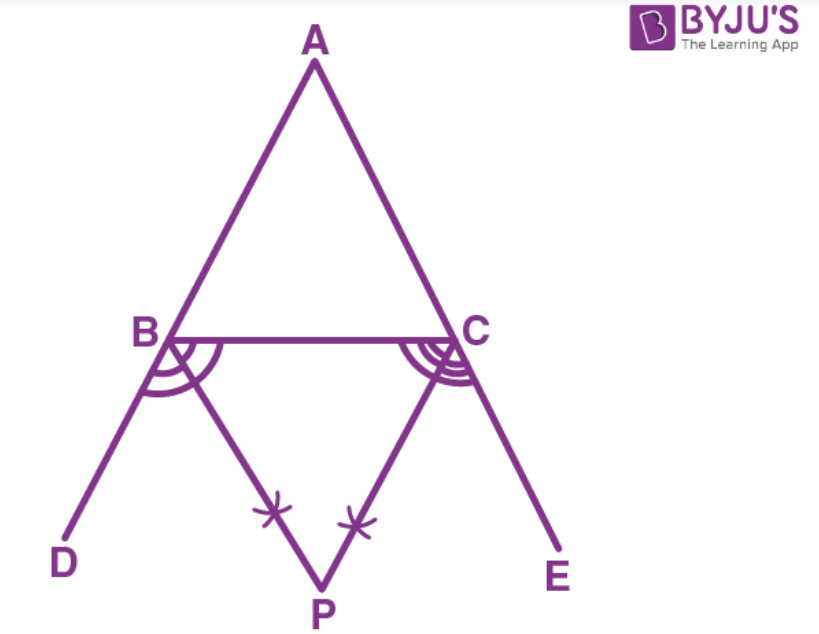In ∆ABC,

AB > AC,

∠ABC < ∠ACB

1800 – ∠ABC > 1800 -∠ACB

(1800 – ∠ABC)/2 > (1800 -∠ABC)/2

900 – ½ ∠ABC > 900 – ½ ∠ACB

∠CBP > ∠BCP (BP is bisector of ∠CBD and CP is bisector of ∠BCE)

PC > PB (side opposite to greater angle is greater)

16. In the following figure; AB is the largest side and BC is the smallest side of triangle ABC.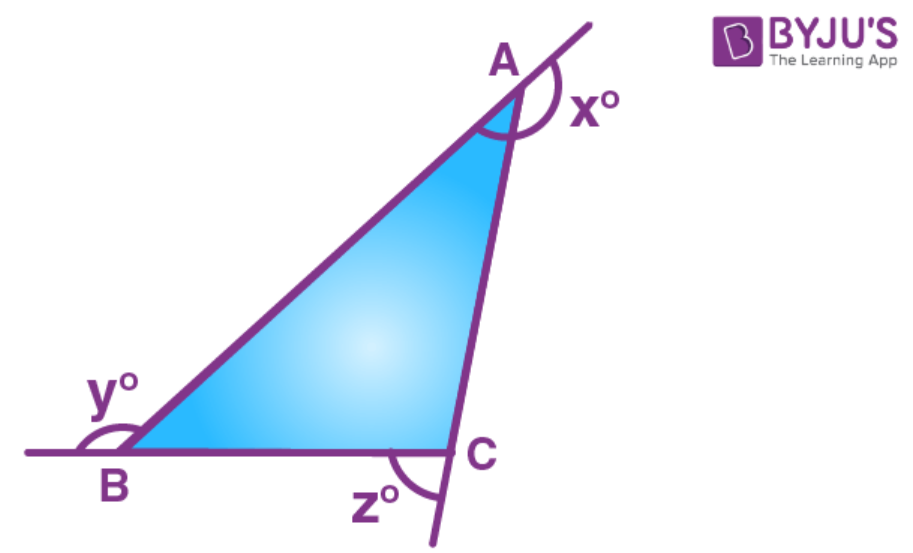Write the angles xo, yo and zo in ascending order of their values.

Solution:

Since AB is the largest side and BC is the smallest side of the triangle ABC

AB > AC > BC

1800 – Z0 > 1800 – y0

-Z0 > -y0 > -x0

Z0 < y0 < x0

17. In quadrilateral ABCD, side AB is the longest and side DC is the shortest.

Prove that:

(i) ∠C > ∠A

(ii) ∠D > ∠B.

Solution: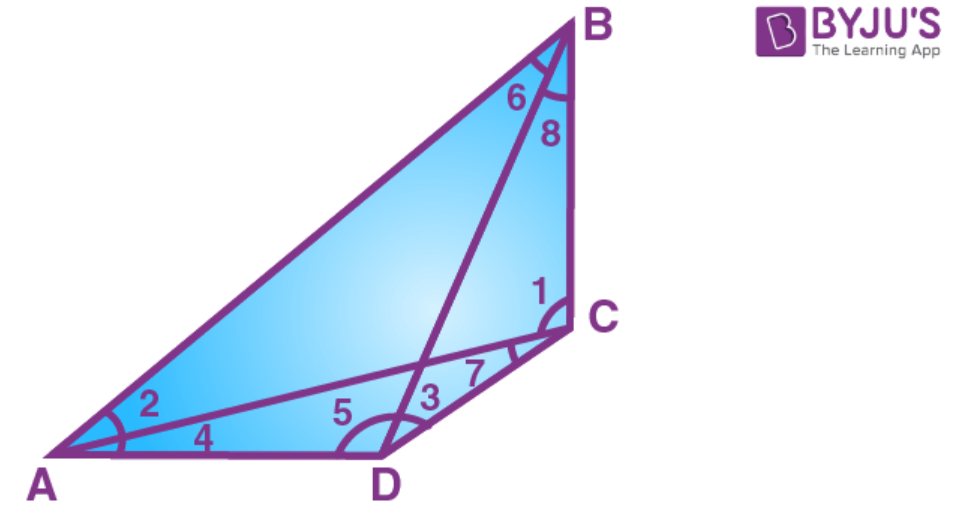Since AB is the longest side and DC is the shortest side.

(i) ∠1 > ∠2 [AB > BC]

∠7 > ∠4 [AD > DC]

∠1 + ∠7 > ∠2 + ∠4

∠C > ∠A

(ii) ∠5 > ∠6[AB > AD]

∠3 > ∠8[BC > CD]

∠5 + ∠3 > ∠6 + ∠8

∠D > ∠B

18. In triangle ABC, side AC is greater than side AB. If the internal bisector of angle A meets the opposite side at point D, prove that: ∠ADC is greater than ∠ADB.

Solution: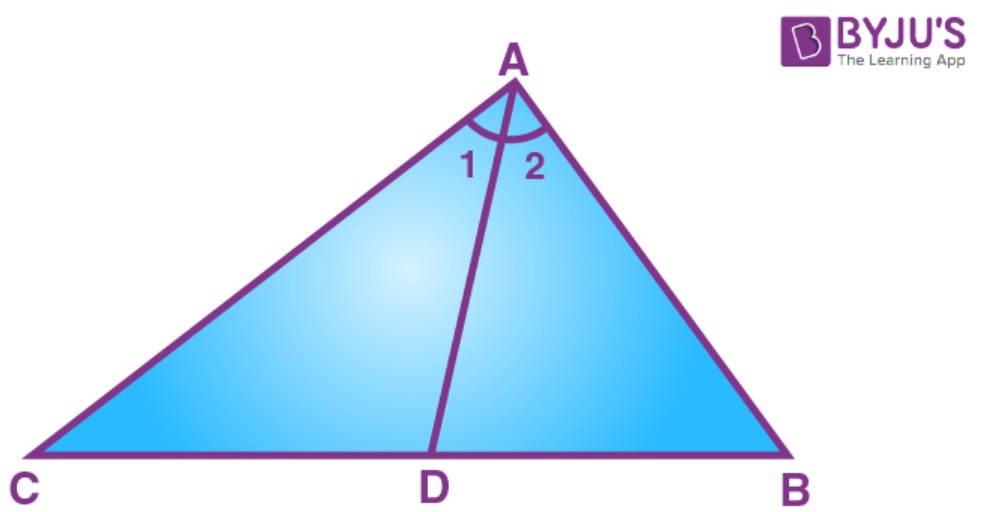But AC > AB [Given]

∠B > ∠C

Also given, ∠2 = ∠1 [AD is bisector of ∠A]

∠2 + ∠B > ∠1 + ∠C ……. (iii)

From (i), (ii) and (iii)

19. In isosceles triangle ABC, sides AB and AC are equal. If point D lies in base BC and point E lies on BC produced (BC being produced through vertex C), prove that:

(ii) AE > AC

Solution: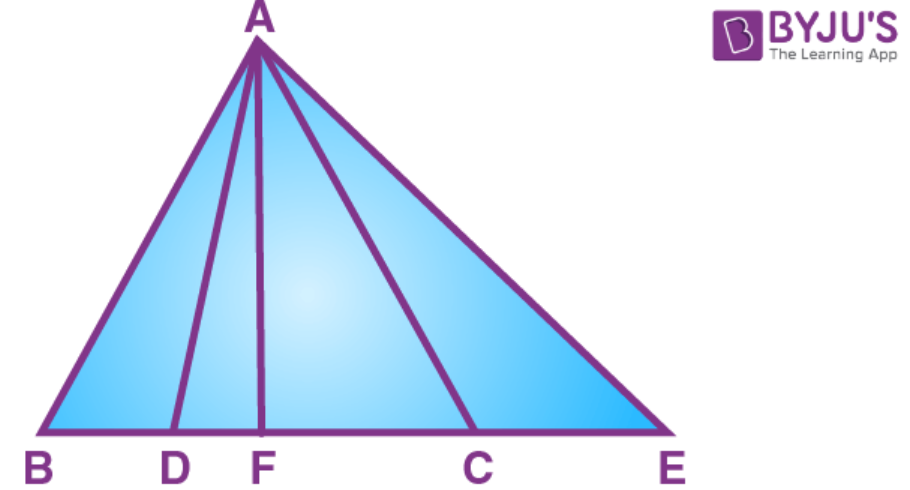We know that the bisector of the angle at the vertex of an isosceles triangle bisects the base at right angle.

Using Pythagoras theorem in ∆ AFB,

AB2 = AF2 + BF2………….. (i)

In ∆ AFD,

AD2 = AF2 + DF2………….. (ii)

We know ABC is isosceles triangle and AB = AC

AC2 = AF2 + BF2 …….. (iii)b[ From (i)]

Subtracting (ii) from (iii)

AC2 – AD2 = AF2 + BF2 – AF2 – DF2

AC2 – AD2 = BF2 – DF2

Let 2DF = BF

AC2 – AD2 = (2DF)2 – DF2

AC2 – AD2 = 4DF2 – DF2

Similarly, AE > AC and AE > AD.

20. Given: ED = EC

Prove: AB + AD > BC.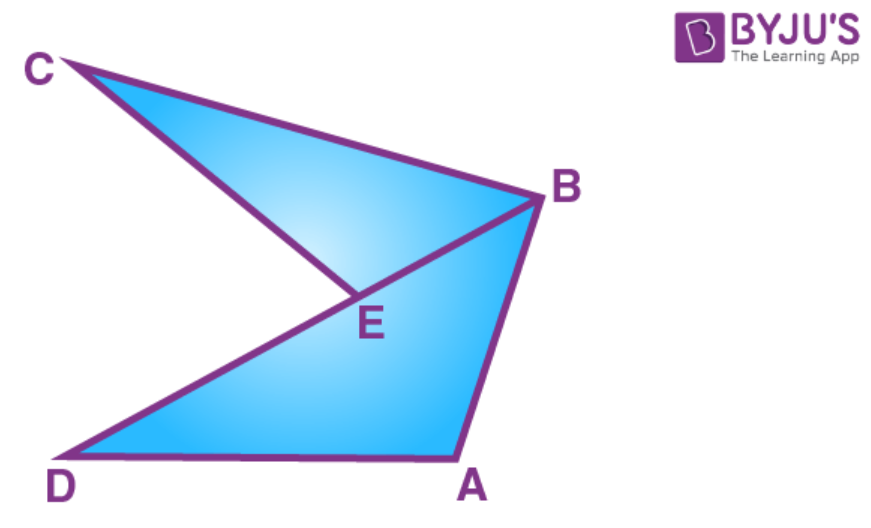Solution:

In ∆CEB

CE + EB > BC

DE + EB > BC (CE = DE)

DB > BC ……… (1)

AD + AB > BD > BC (from 1)

21. In triangle ABC, AB > AC and D is a point in side BC. Show that: AB > AD

Solution: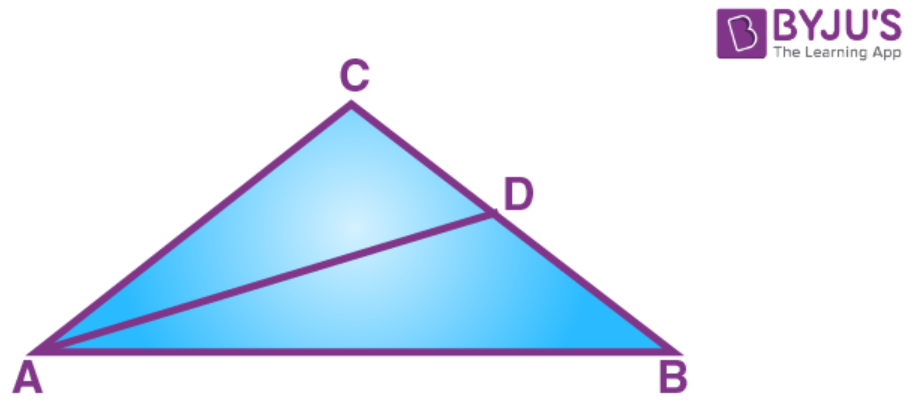Given that AB > AC

∠C > ∠B ………. (1)

∠ADB = ∠DAC + ∠C (exterior angle)

∠ADB > ∠C > ∠B (from 1)

## Selina Solutions for Class 9 Maths Chapter 11- Inequalities

The Chapter 11, Inequalities, is composed of 1 exercise and the solutions given here contain answers for all the questions present in this exercise. Let us have a look at some of the topics that are being discussed in this chapter.

11.1 Introduction

11.2 Theorems:

1. If two sides of a triangle are unequal, the greater side has the greater angle opposite to it.
2. If two angles of a triangle are unequal, the greater angle has the greater side opposite to it.
3. Of all the lines, that can be drawn to a given straight line from a given point outside it, the perpendicular is the shortest.

## Selina Solutions for Class 9 Maths Chapter 11- Inequalities

The Chapter 11 of class 9 takes the students to the topic of Inequalities related to triangles. In chapter 10, students learn about the properties and problems related to isosceles triangle, a triangle with 2 sides and 2 angles equal. However, in chapter 11, students learn about the inequalities related to triangles.Read and learn the Chapter 11 of Selina textbook to familiarize with the concepts related to Inequalities. Learn the Selina Solutions for Class 9 effectively to score high in the examination.